# NCERT Exemplar Class 8 Maths Solutions for Chapter 1 - Rational Numbers

## NCERT Exemplar Solutions Class 8 Maths Chapter 1 – Free PDF Download

The NCERT Exemplar Class 8 Maths Chapter 1 Rational Numbers is provided here for students to prepare for exams. These exemplar problems and solutions are designed by the subject experts in accordance with the CBSE syllabus (2023-2024) for 8th standard, which covers all the topics of Class 8 Maths Chapter 1. In this chapter, students will learn about the properties of rational numbers like closure, commutativity, associativity, negative of a number and rational numbers between two numbers. Solving Exemplar Questions for Class 8 will help students to grasp the fundamental concepts of the chapter. Below is the list of topics covered in Chapter 1 of Class 8 Maths subject.

• Properties of rational numbers, whole numbers and integers
• Closure, commutativity and associativity properties
• Some more properties, such as the role of zero (0), the role of 1, the negative of a number, and reciprocal
• Distributivity of multiplication over addition for rational numbers
• Number line representation of rational numbers
• Finding rational numbers between two rational numbers

Solve these exemplars problems to score good marks in the final exam. Also, it is recommended to solve sample papers and previous years’ question papers to get an idea of the question types asked in the annual exam from Chapter 1 Rational Numbers. BYJU’S also provides notes, exemplar books, NCERT Solutions and question papers to help students practise well for their exams.

### NCERT Exemplar Class 8 Maths Solutions Chapter 1 Rational Numbers:-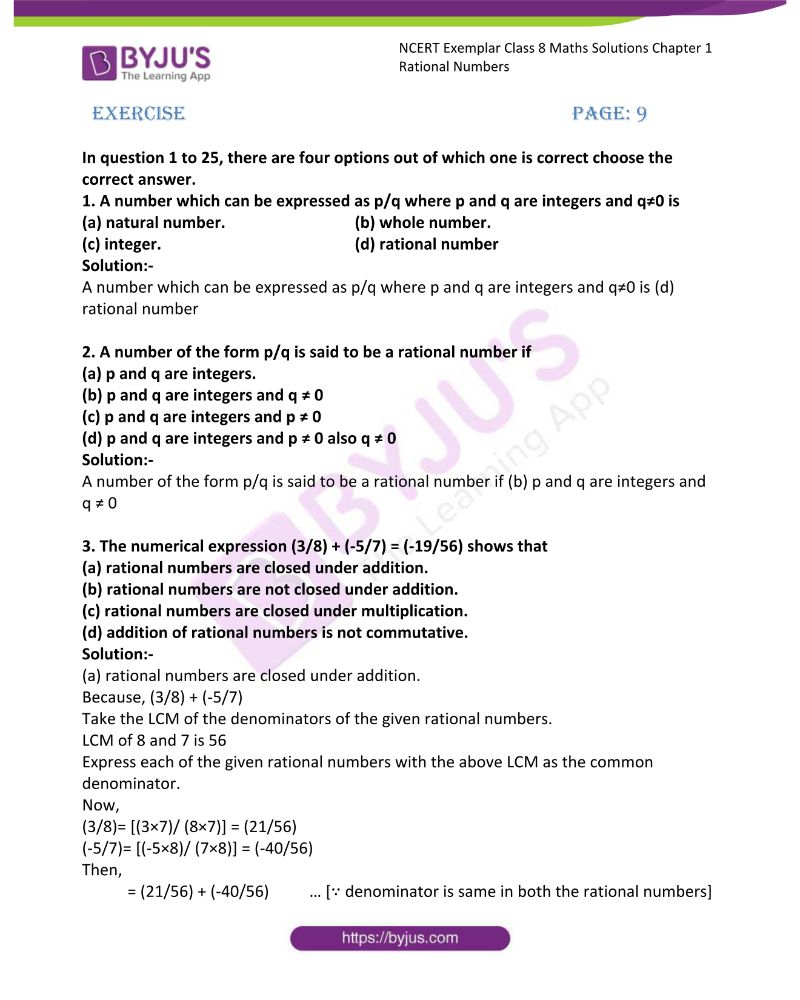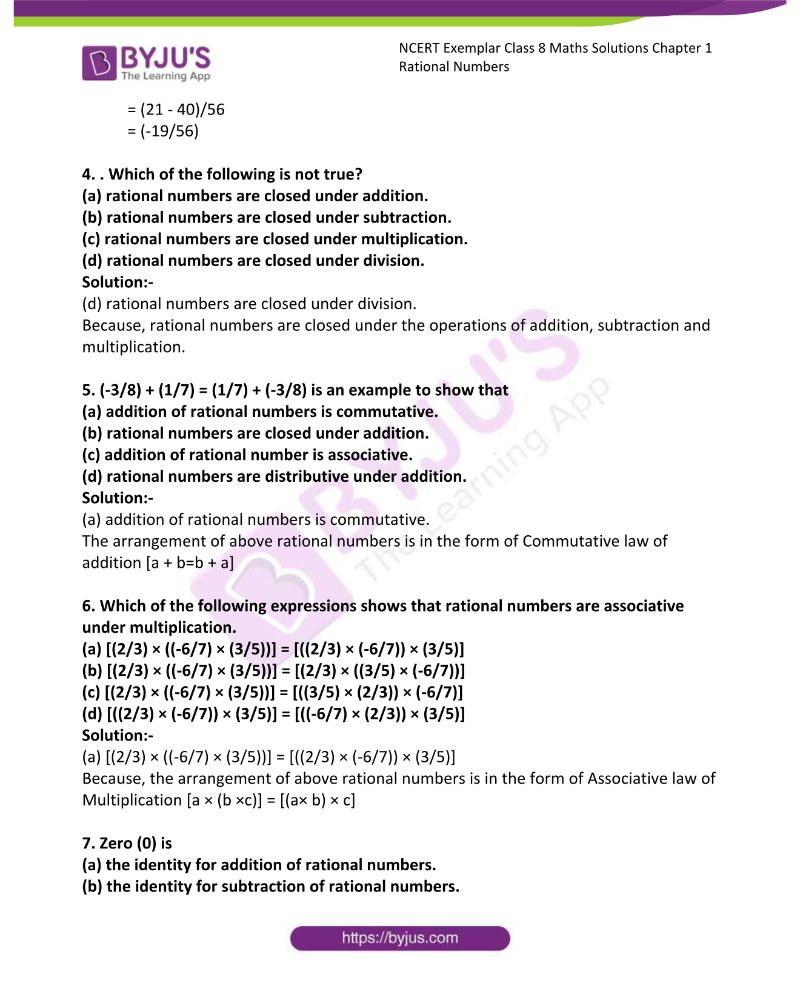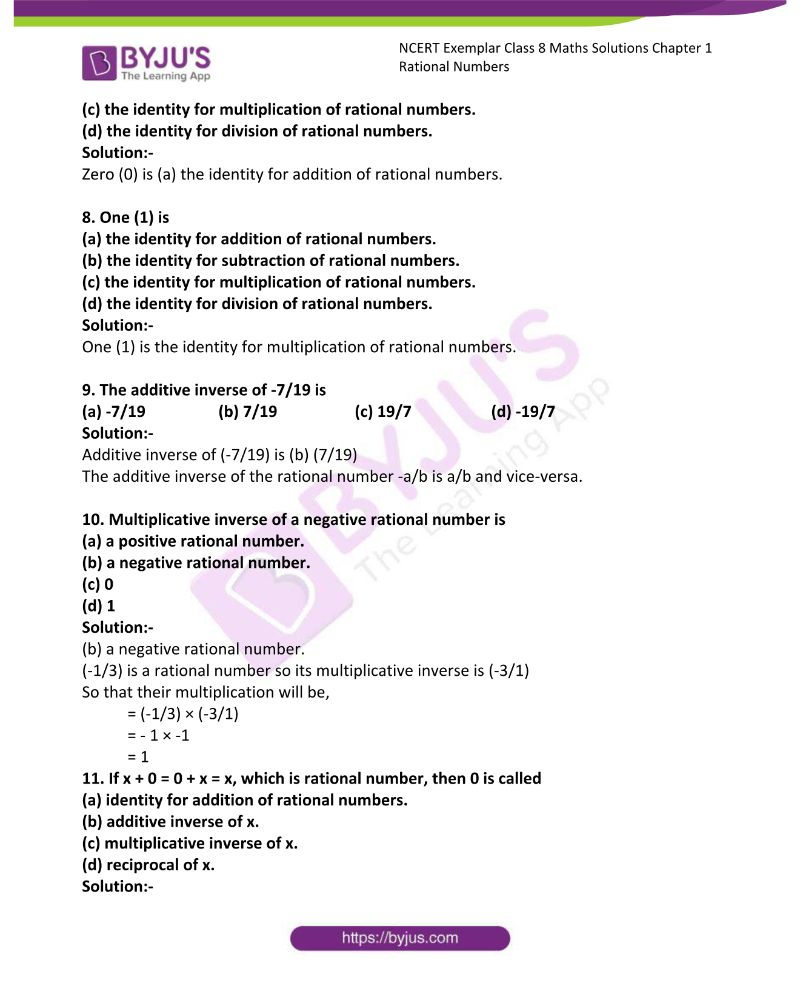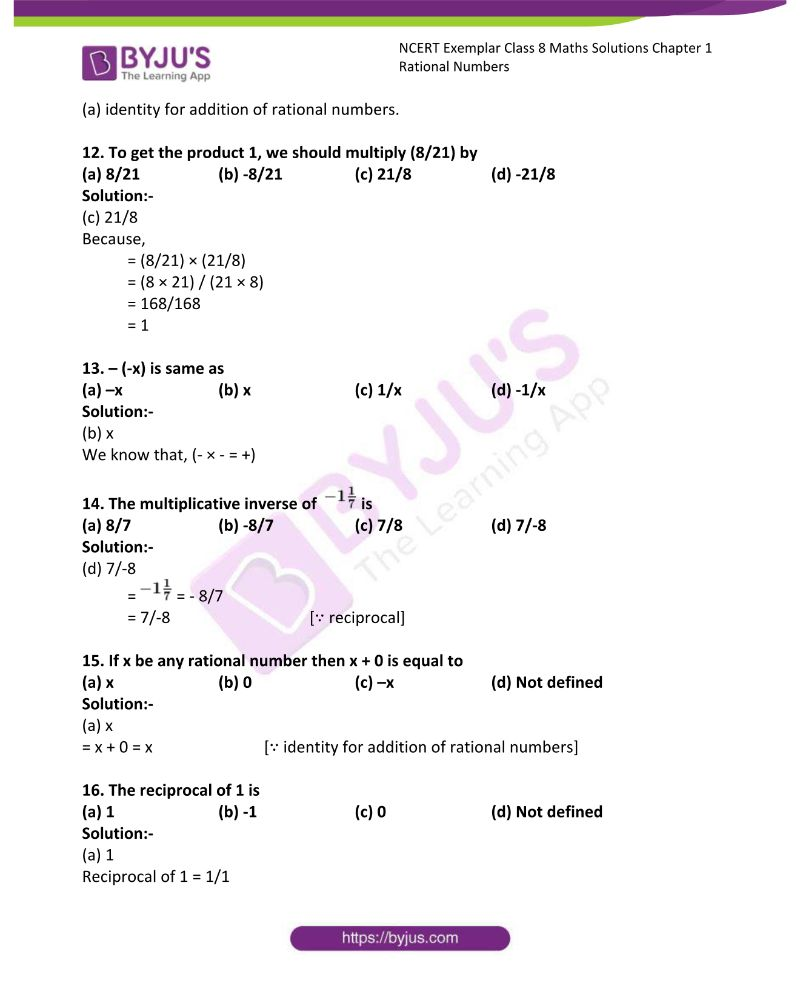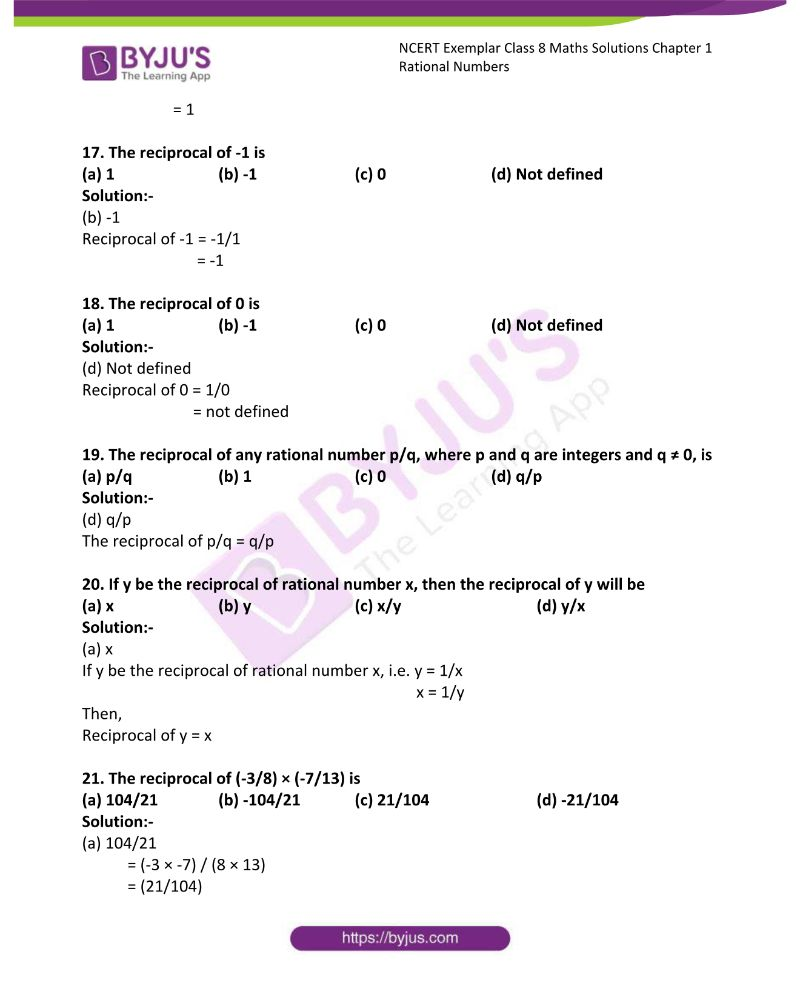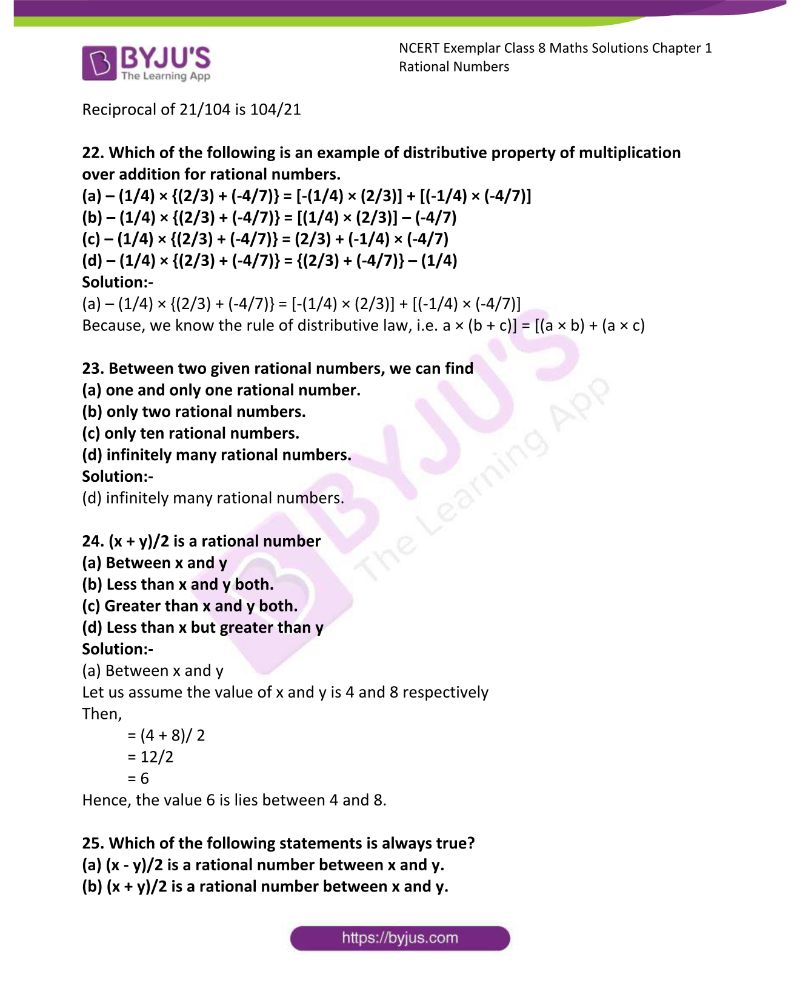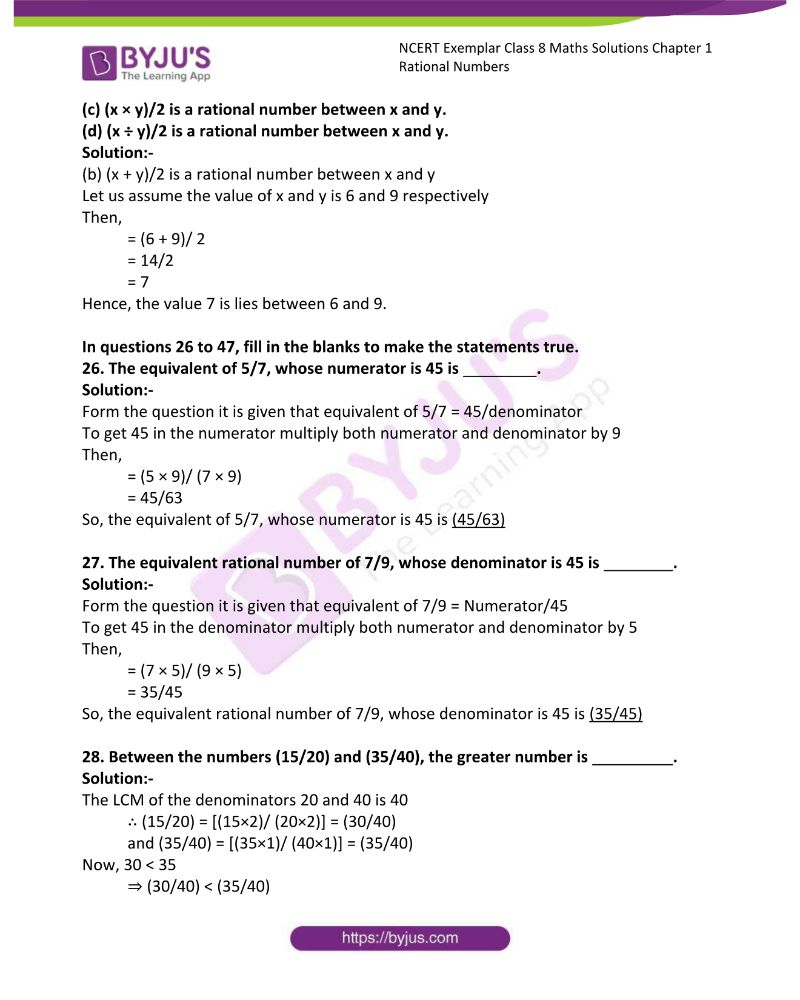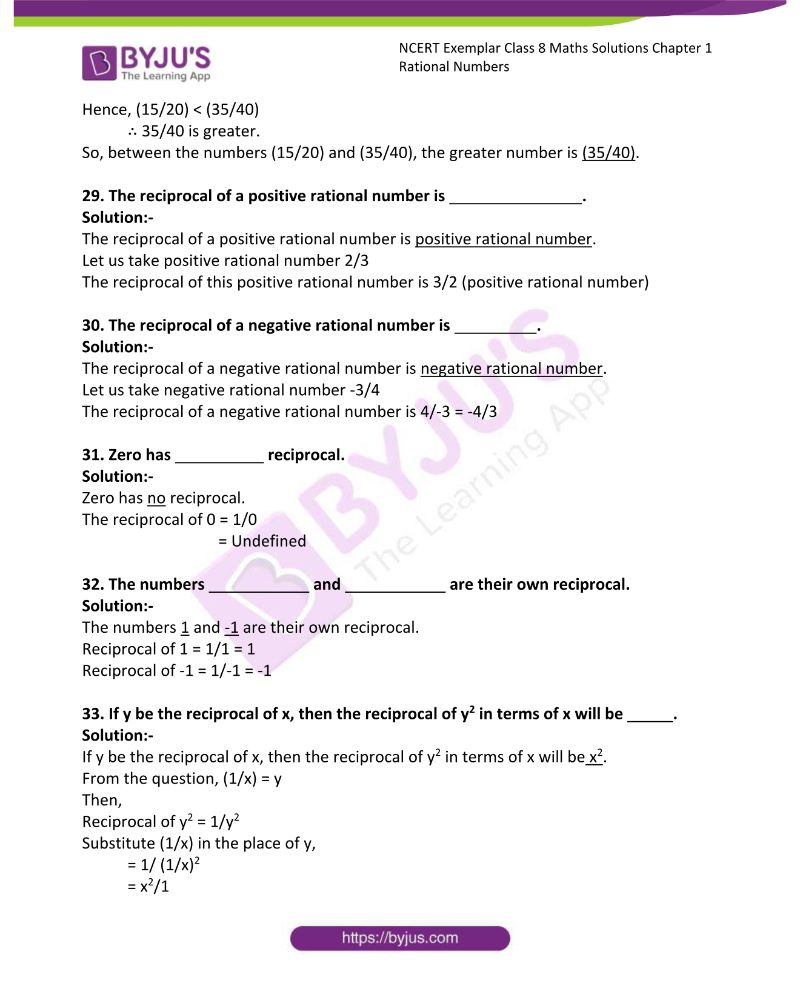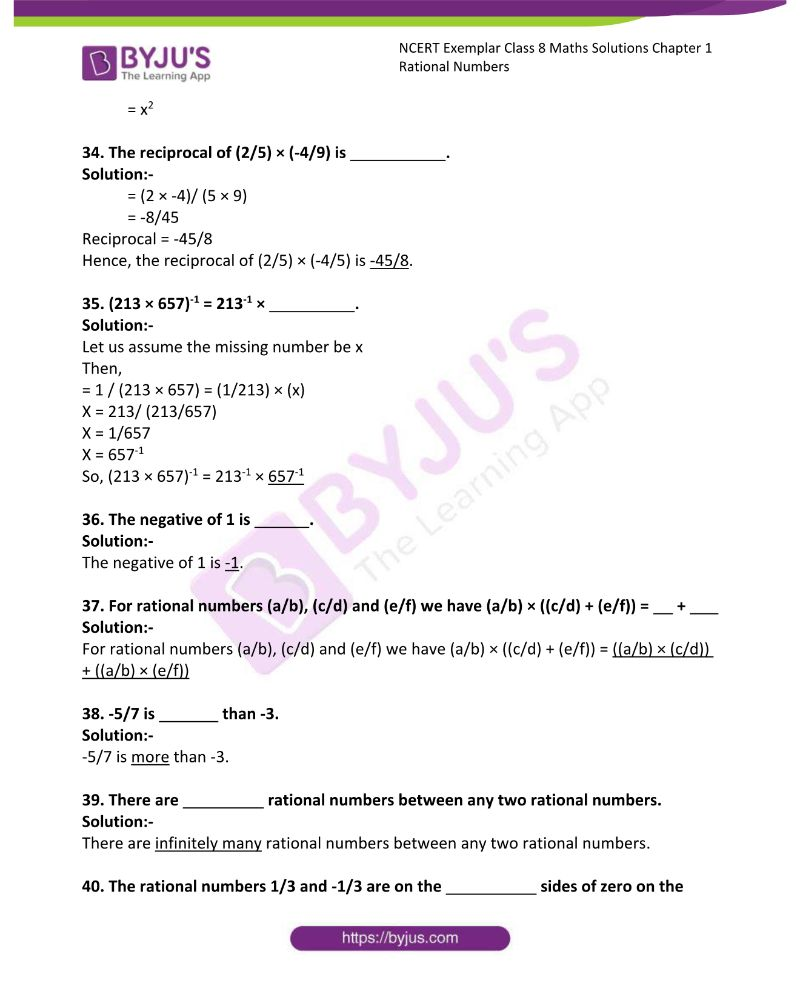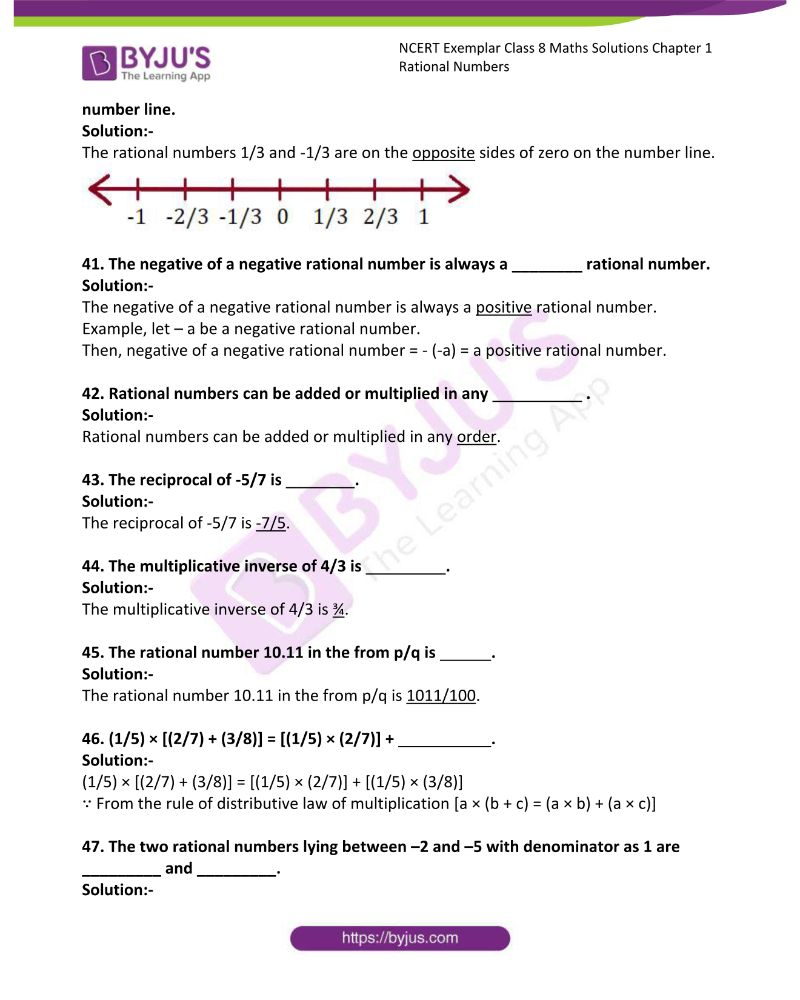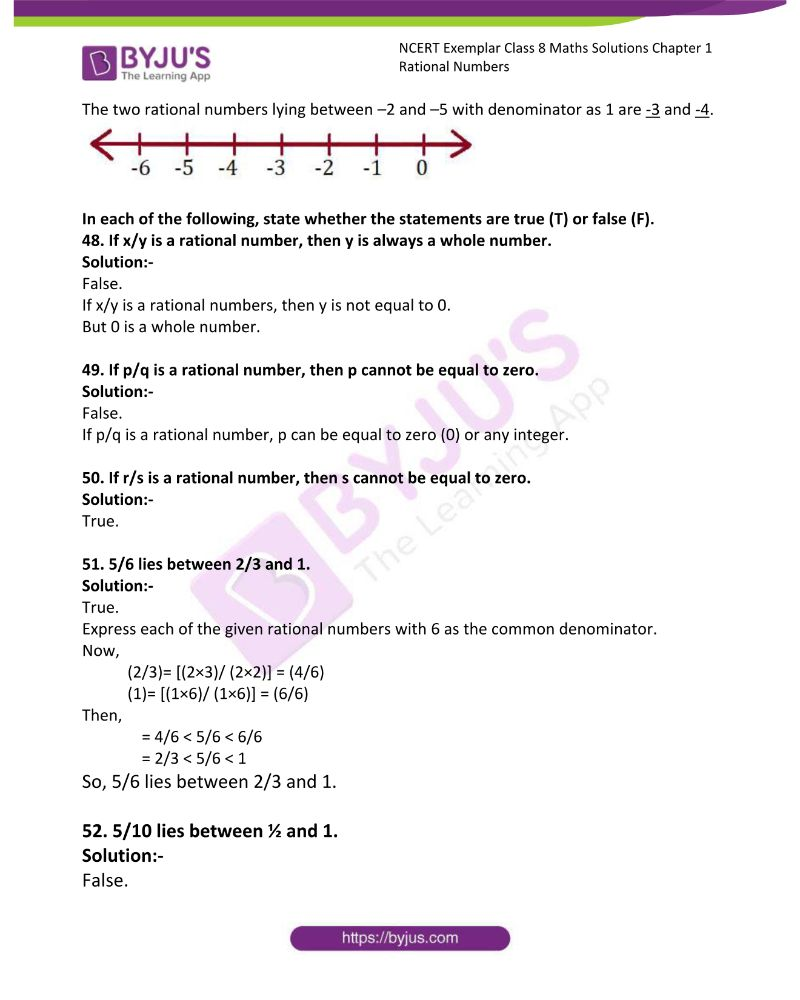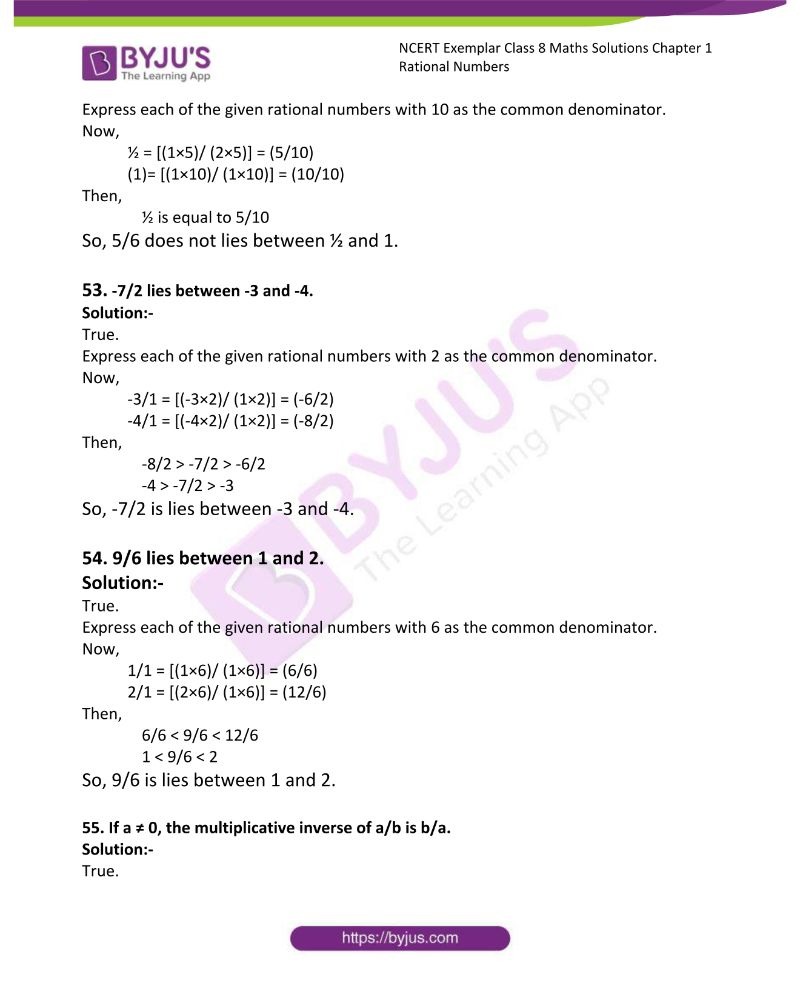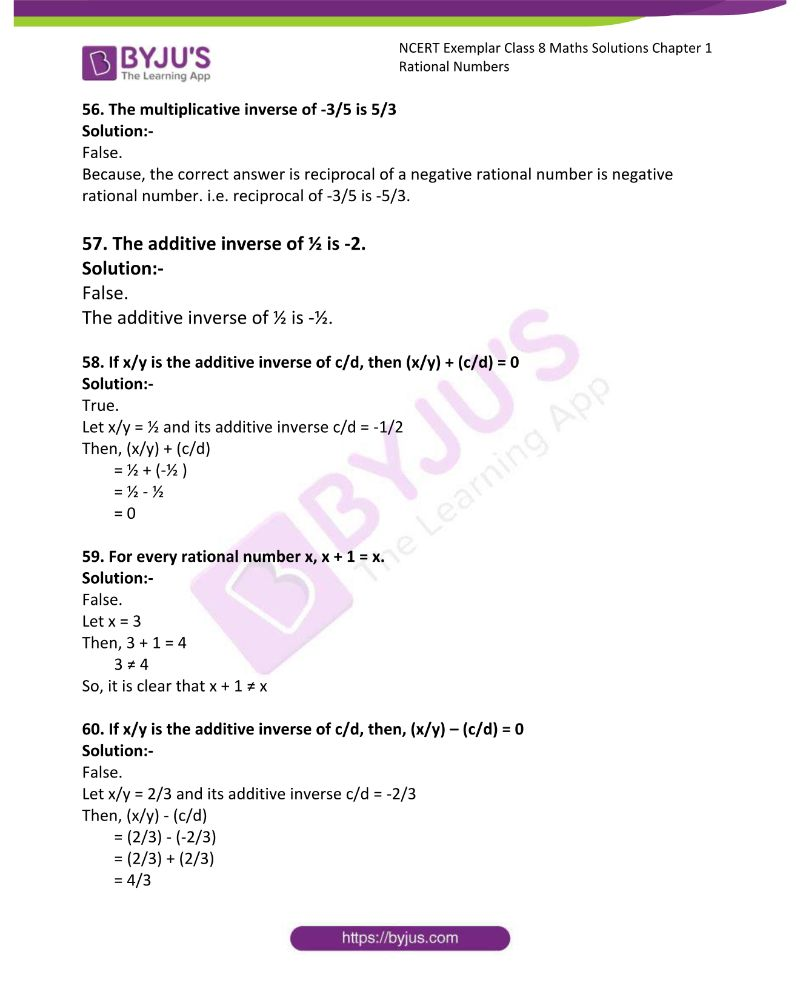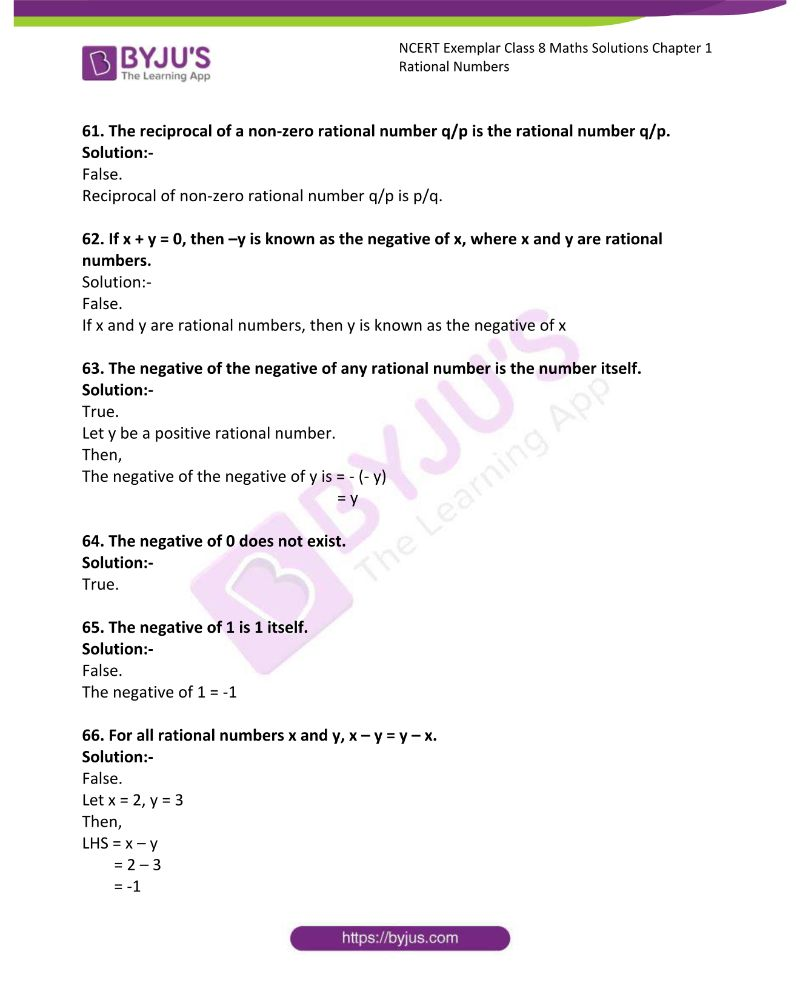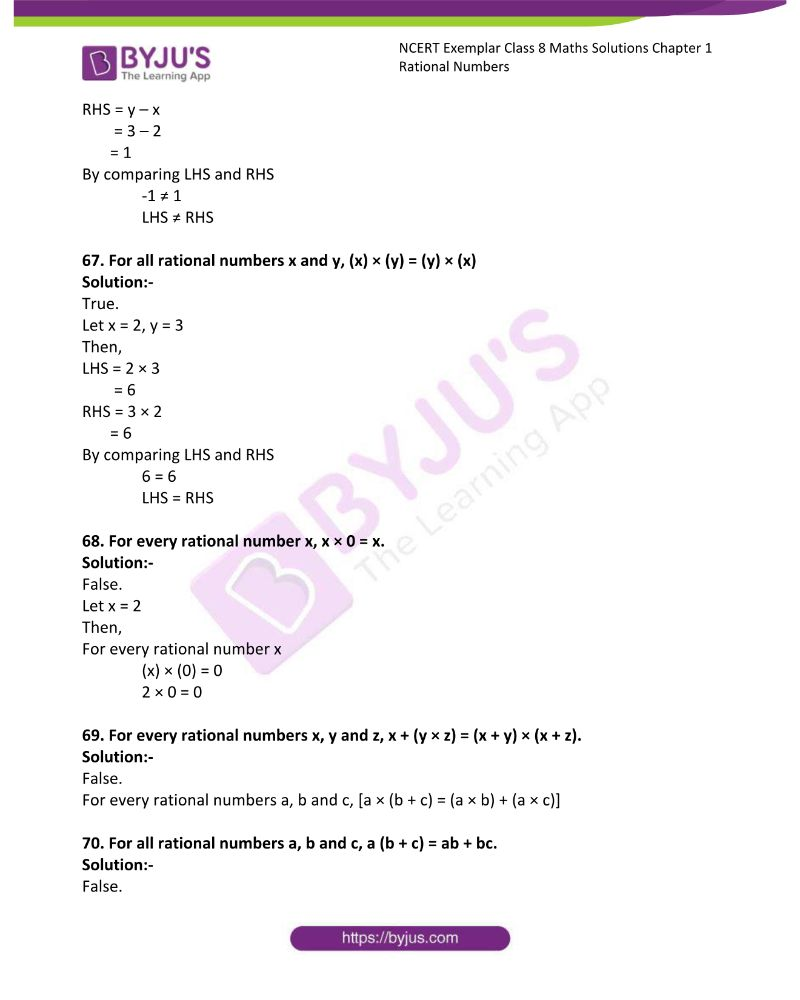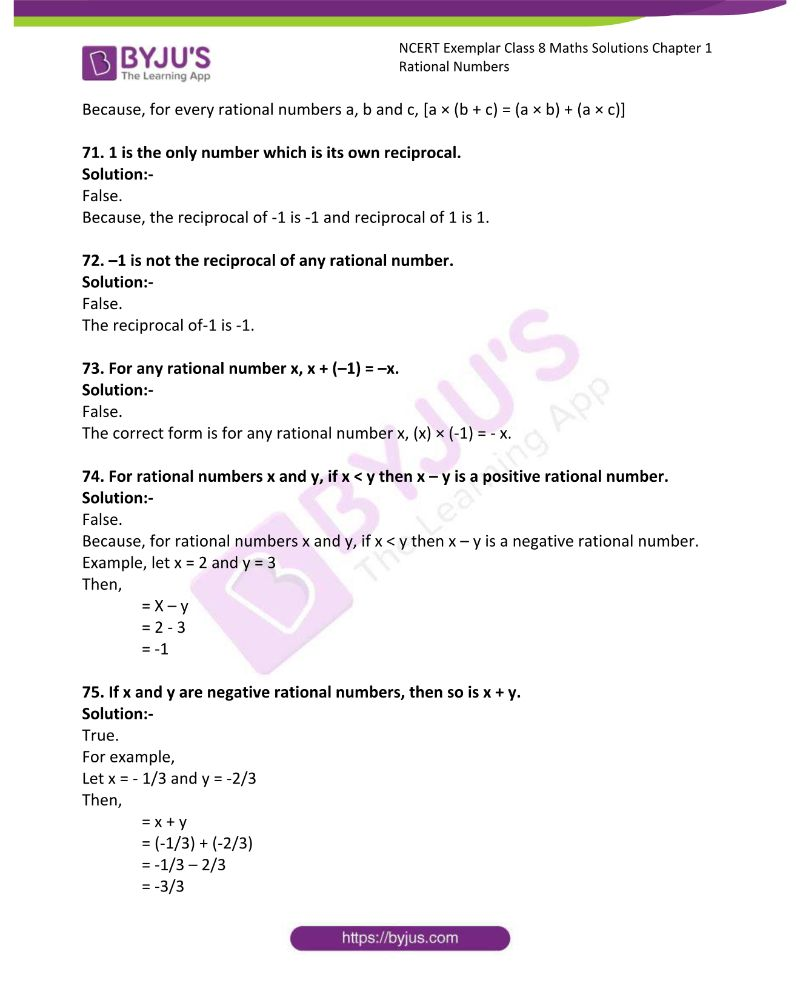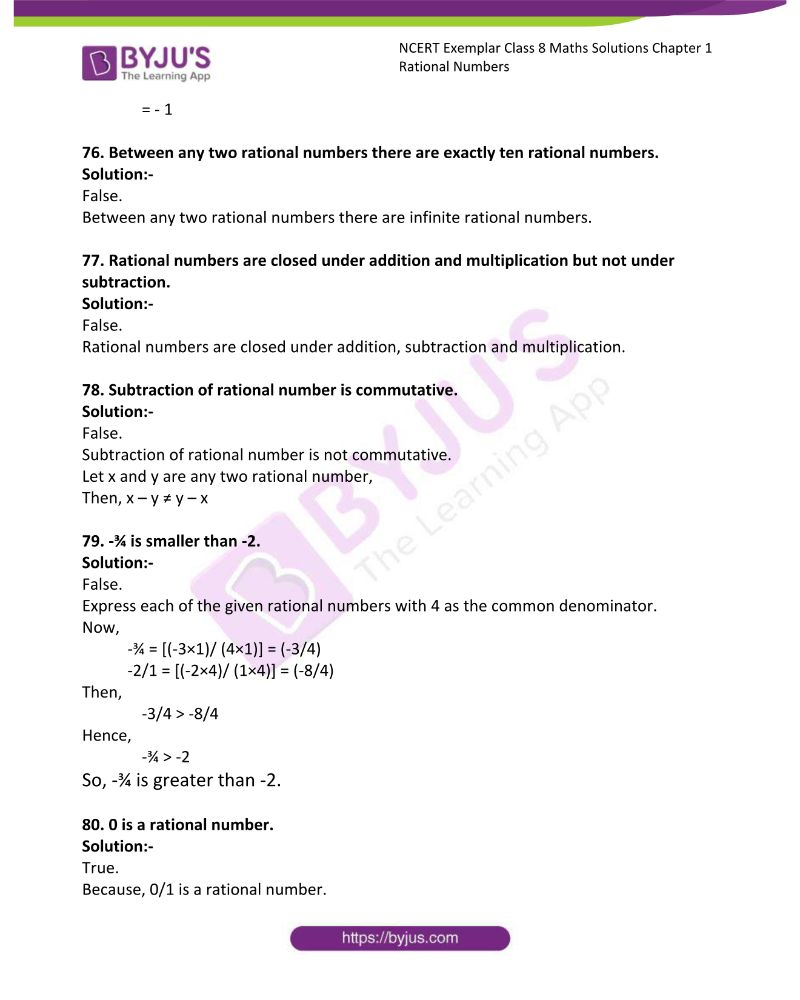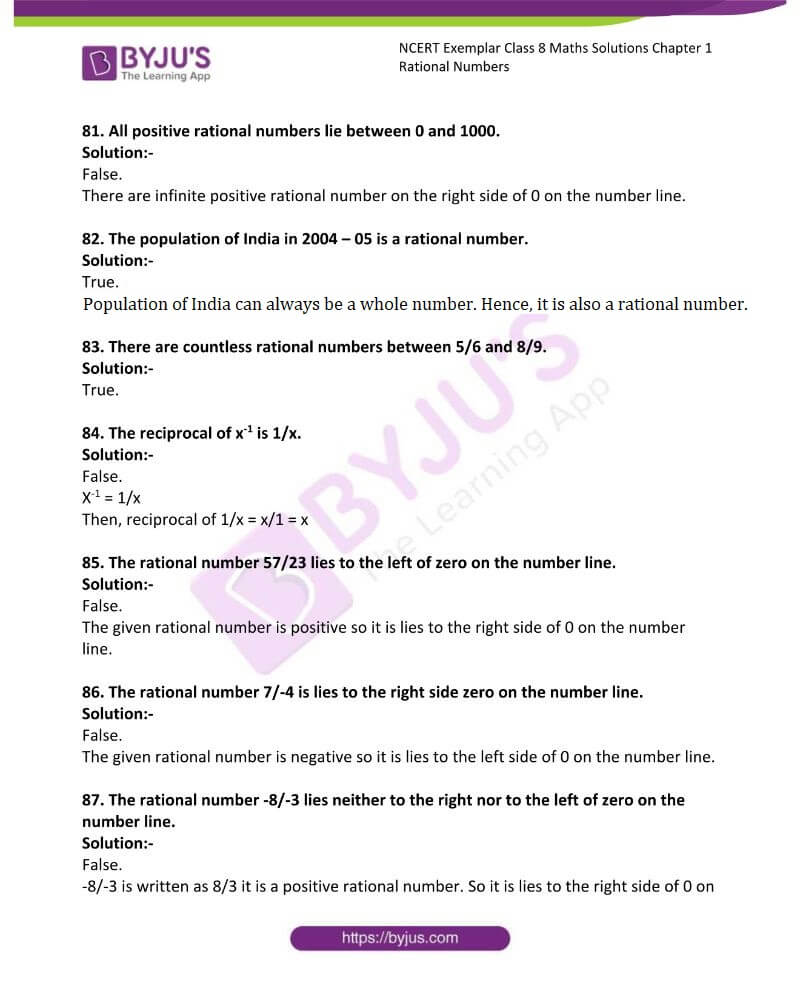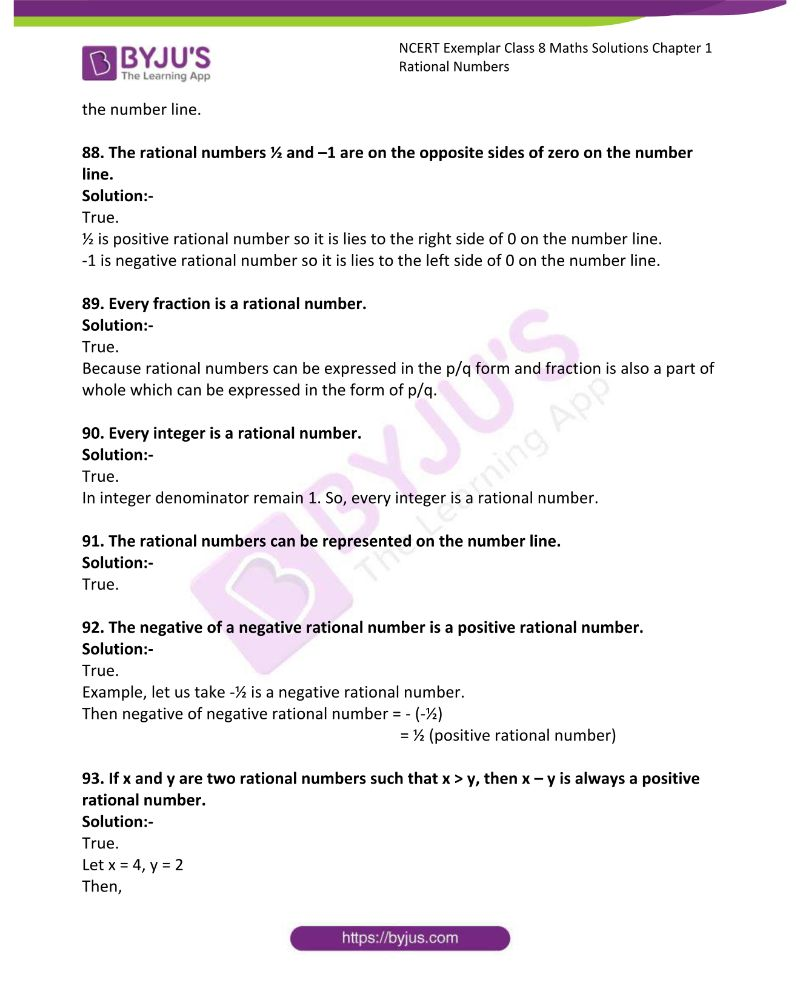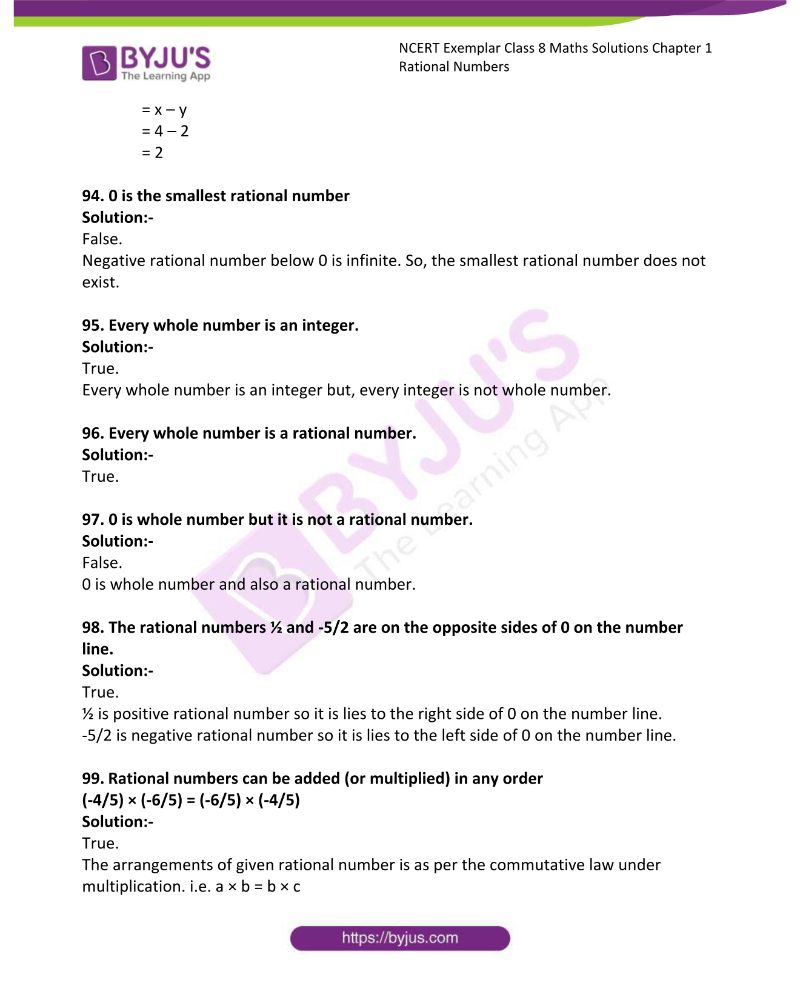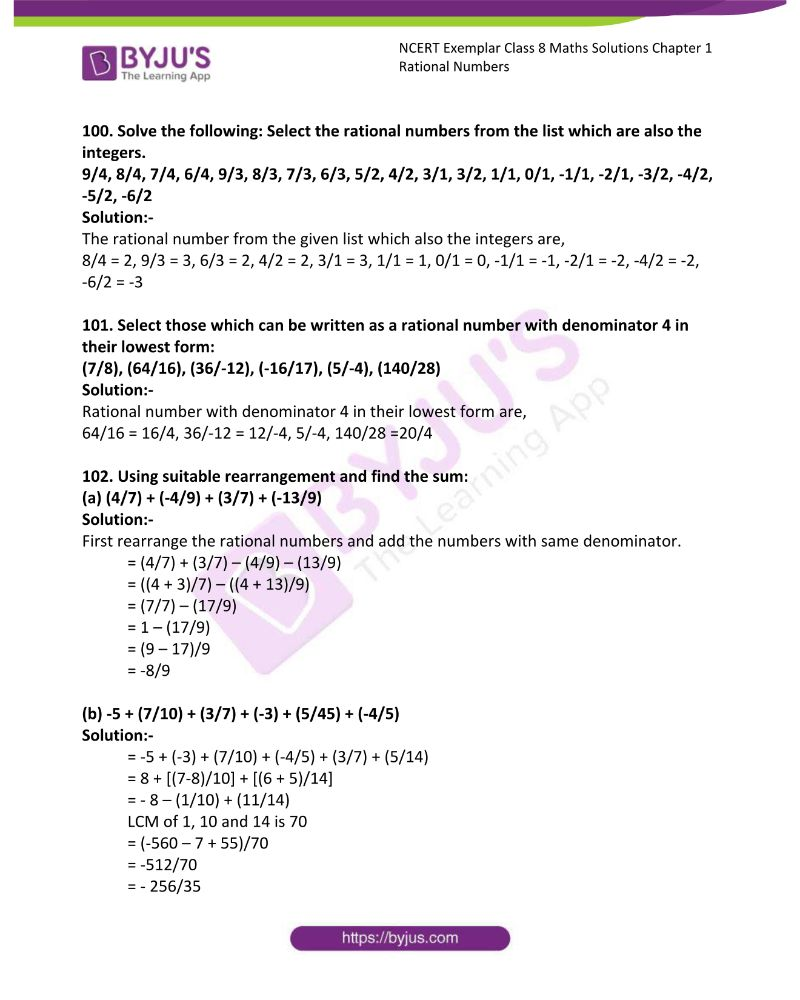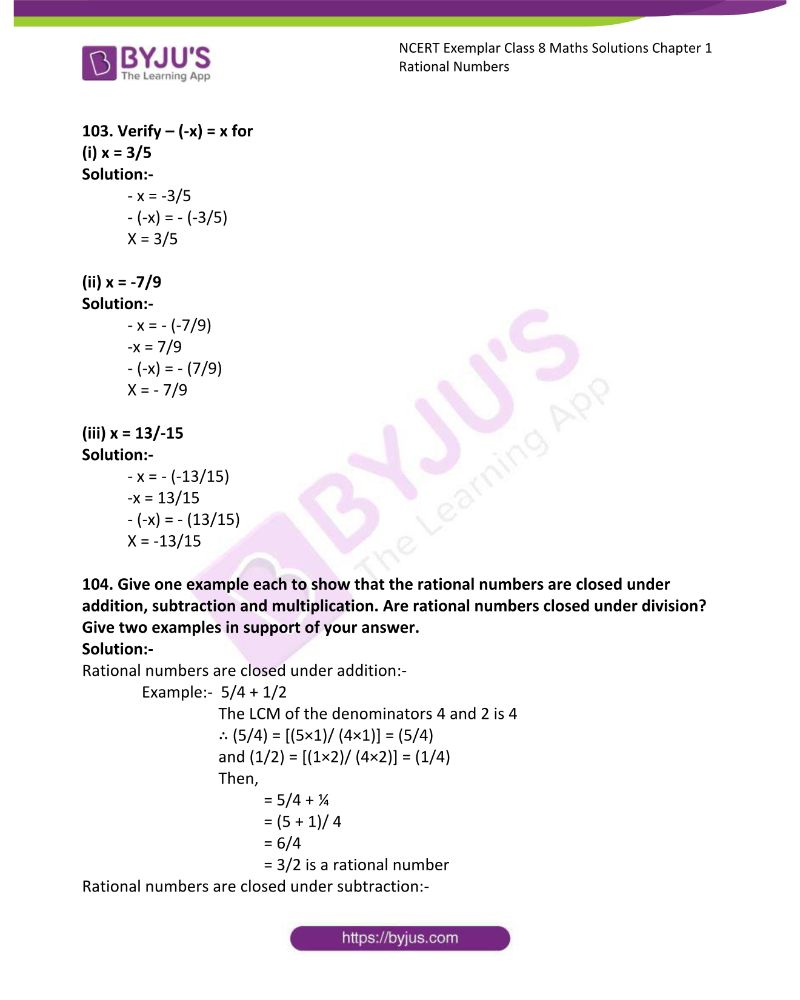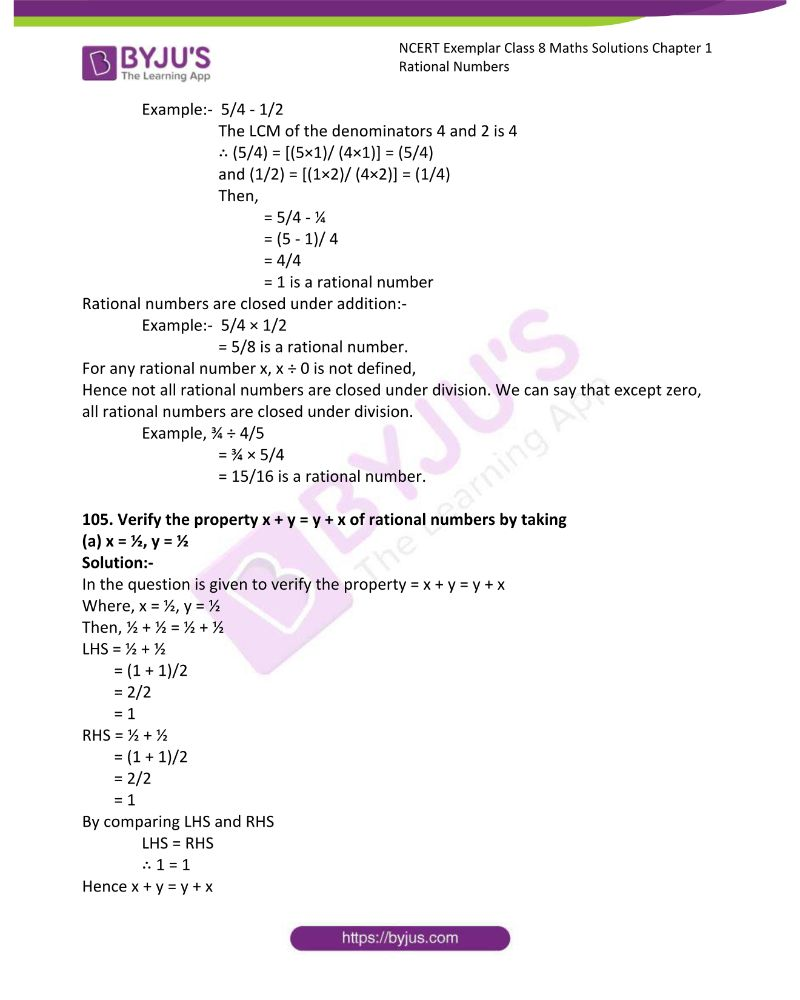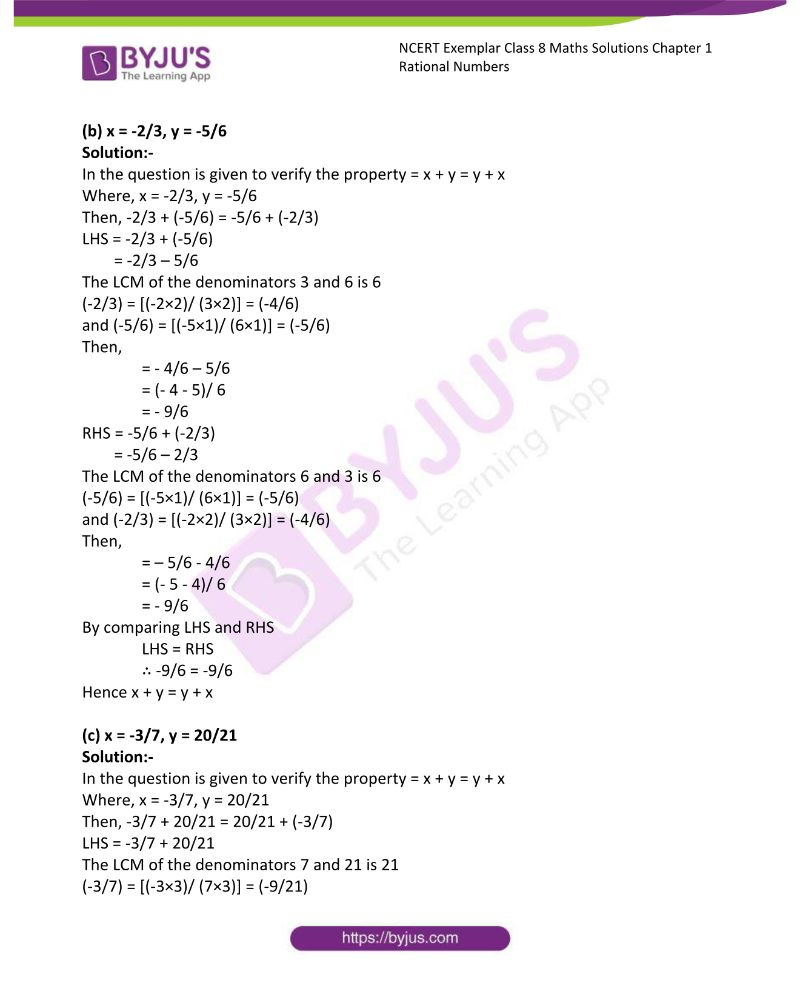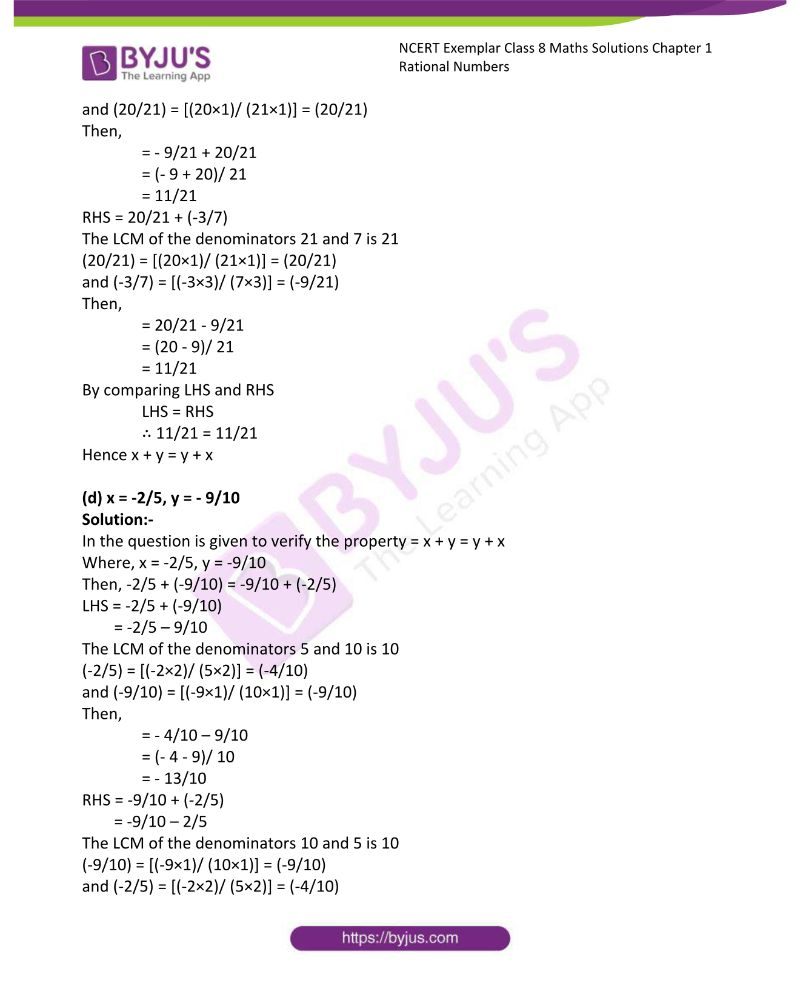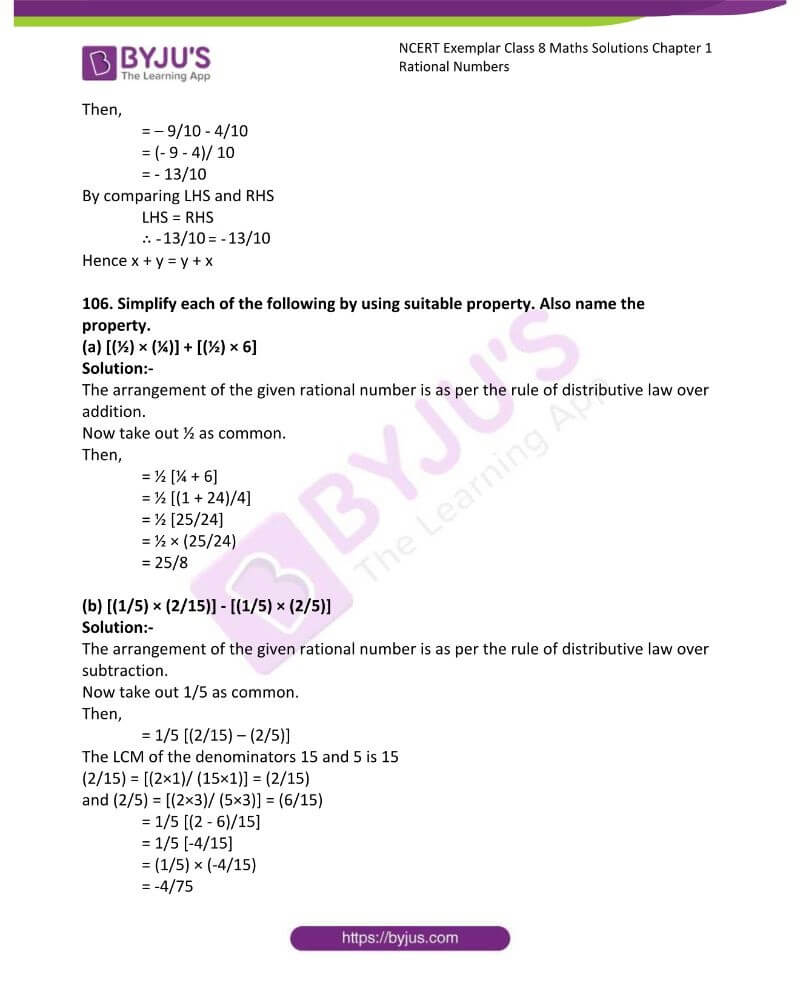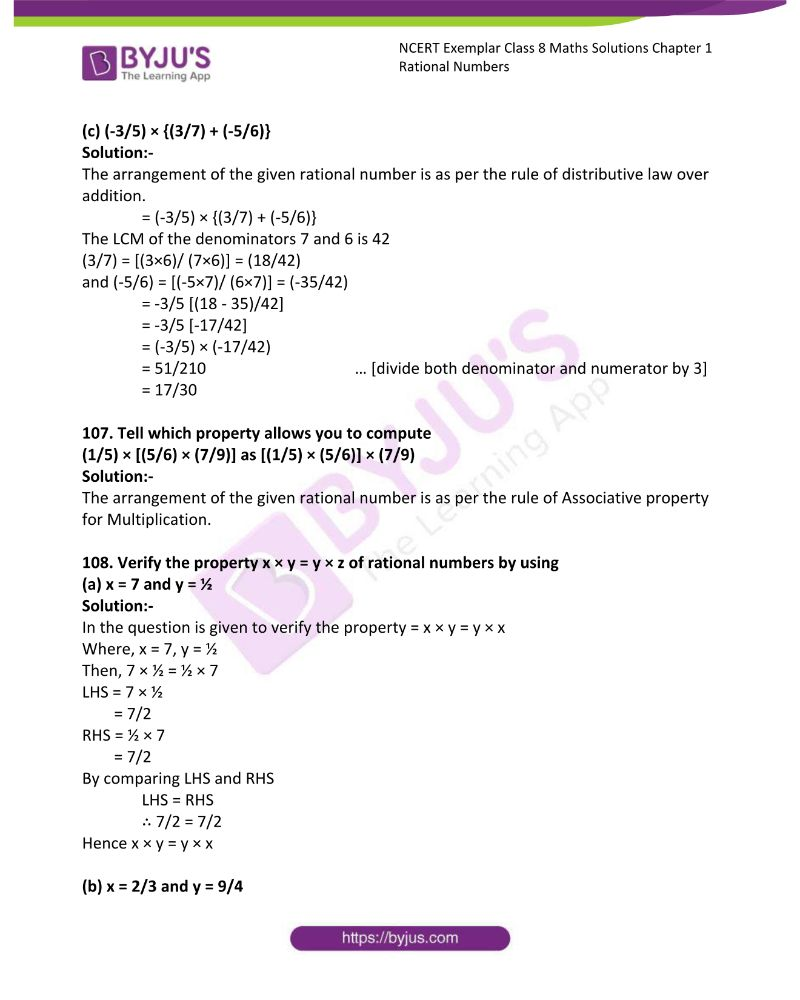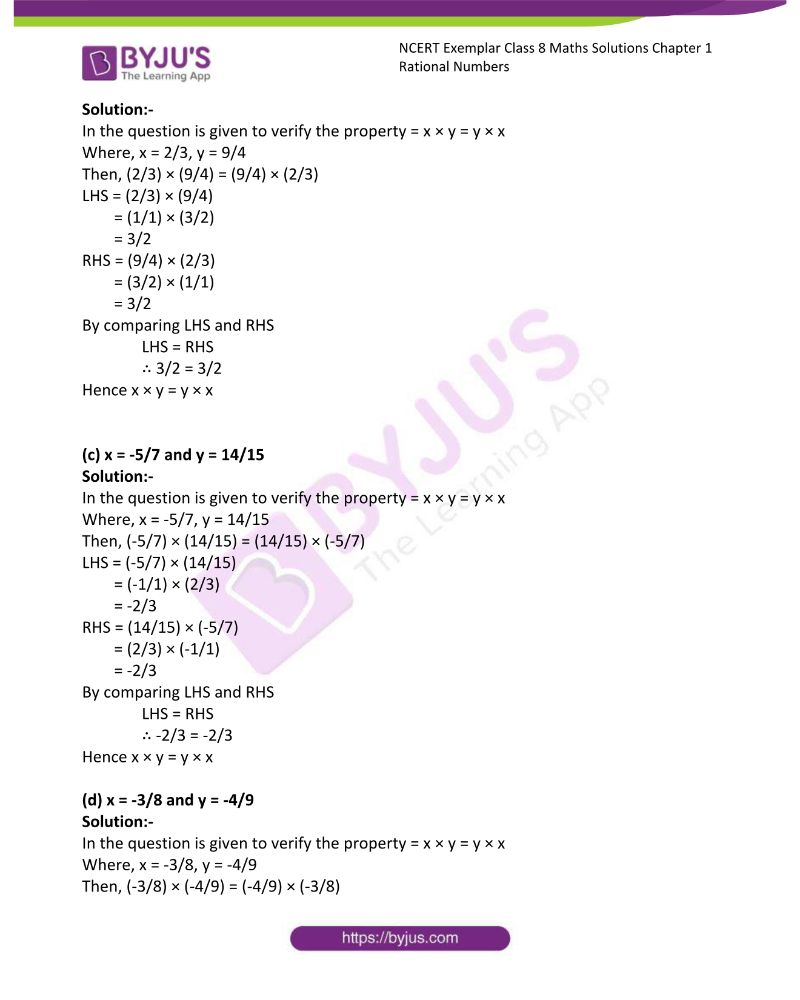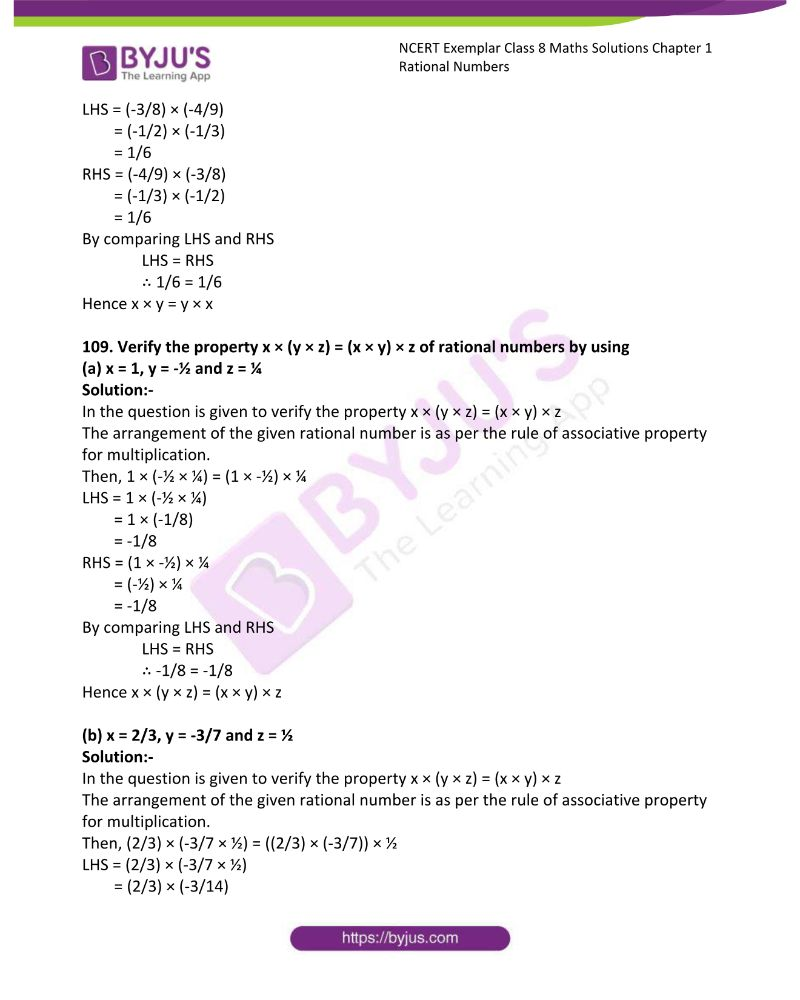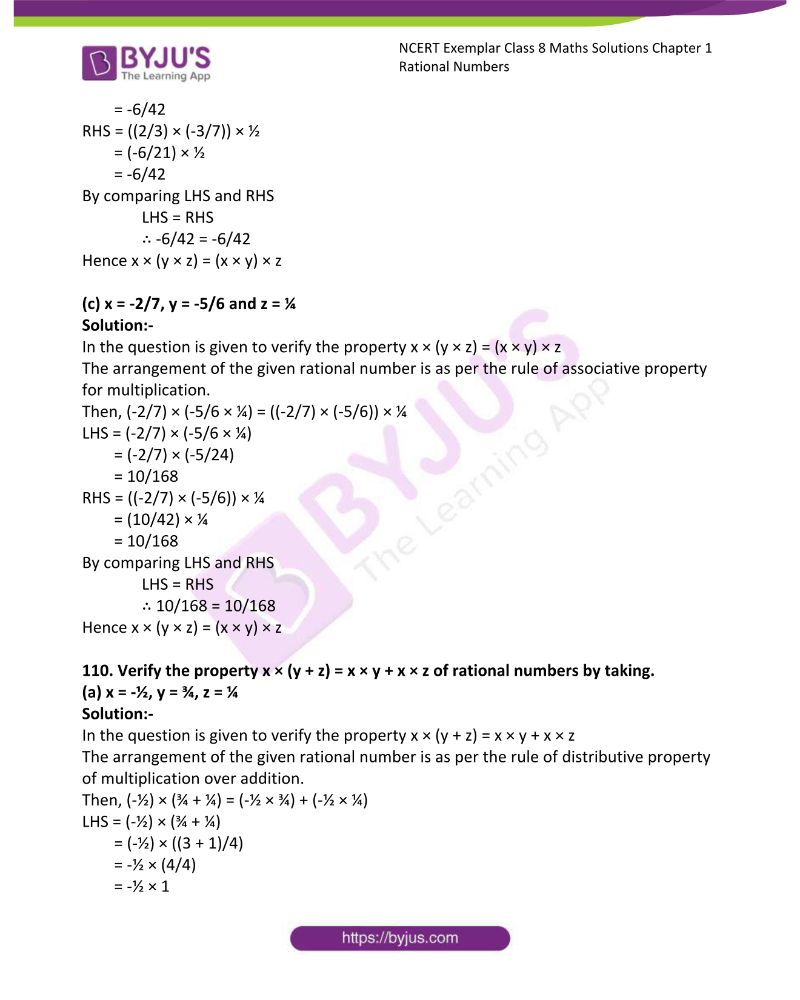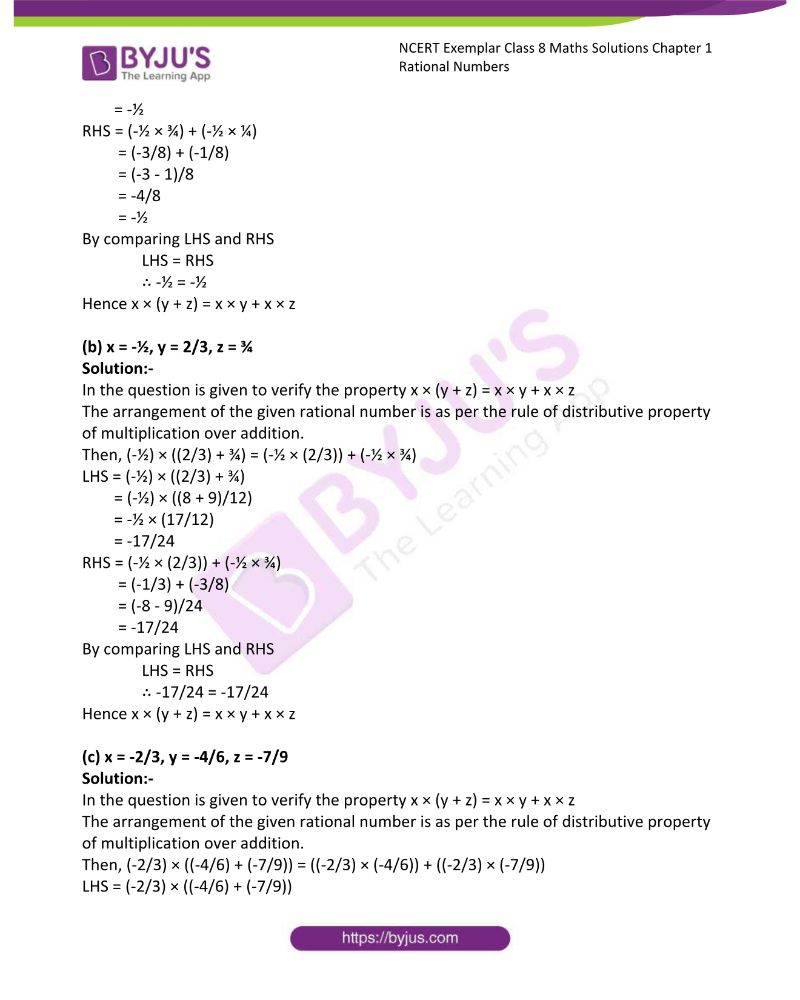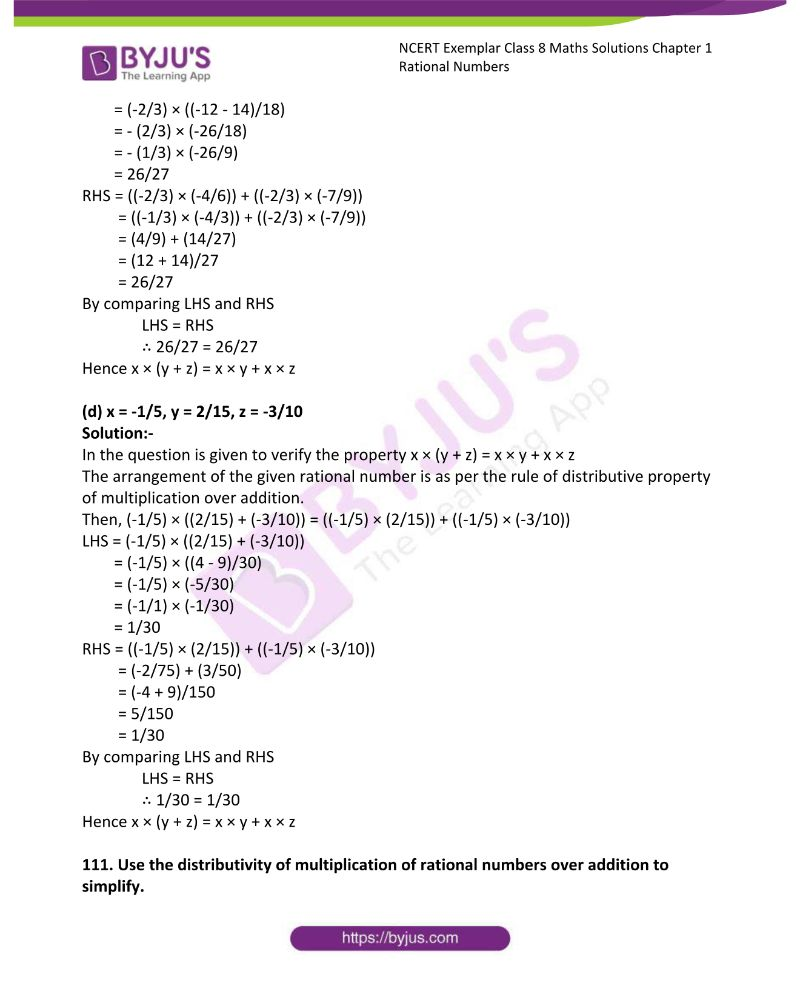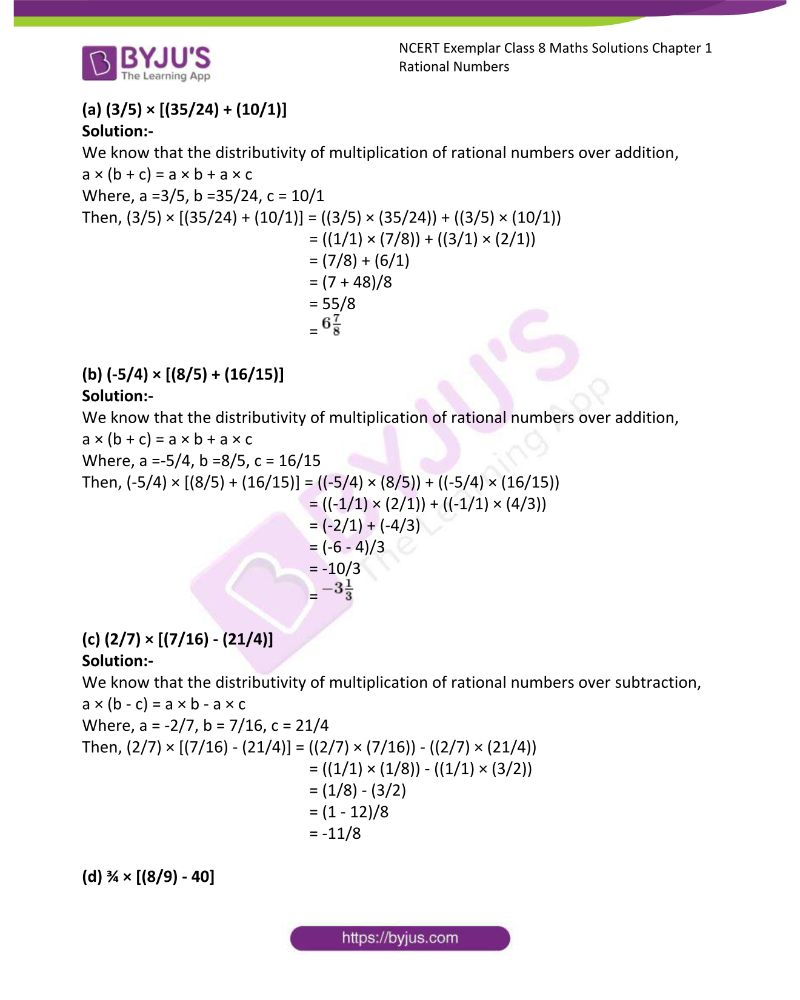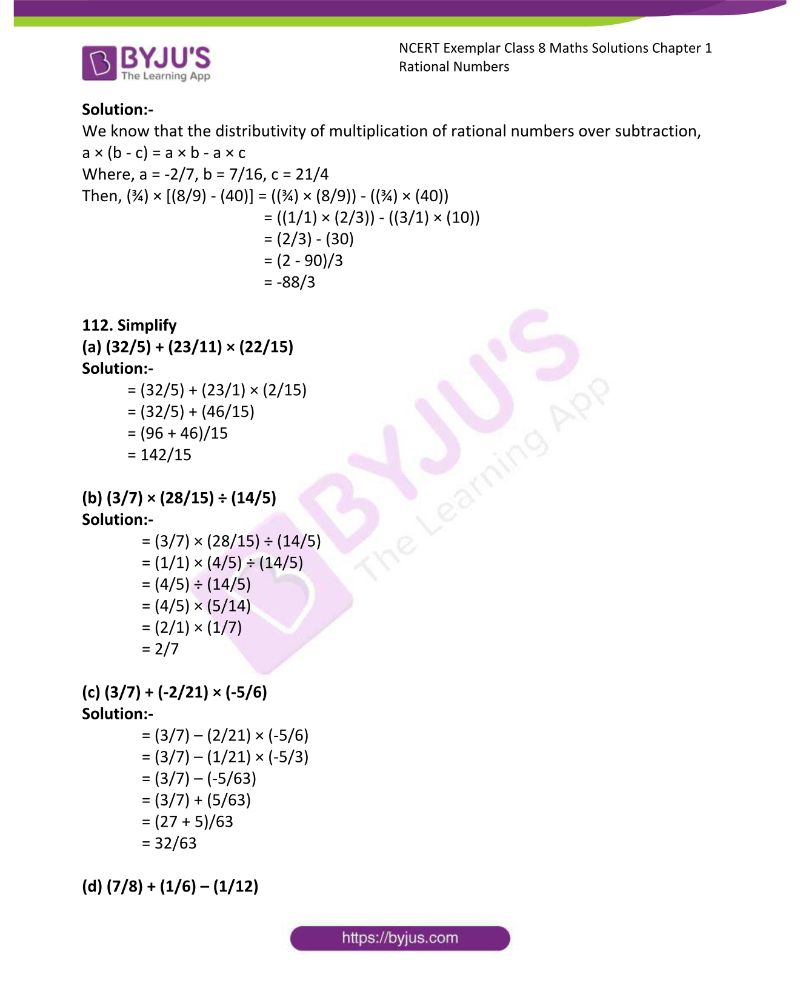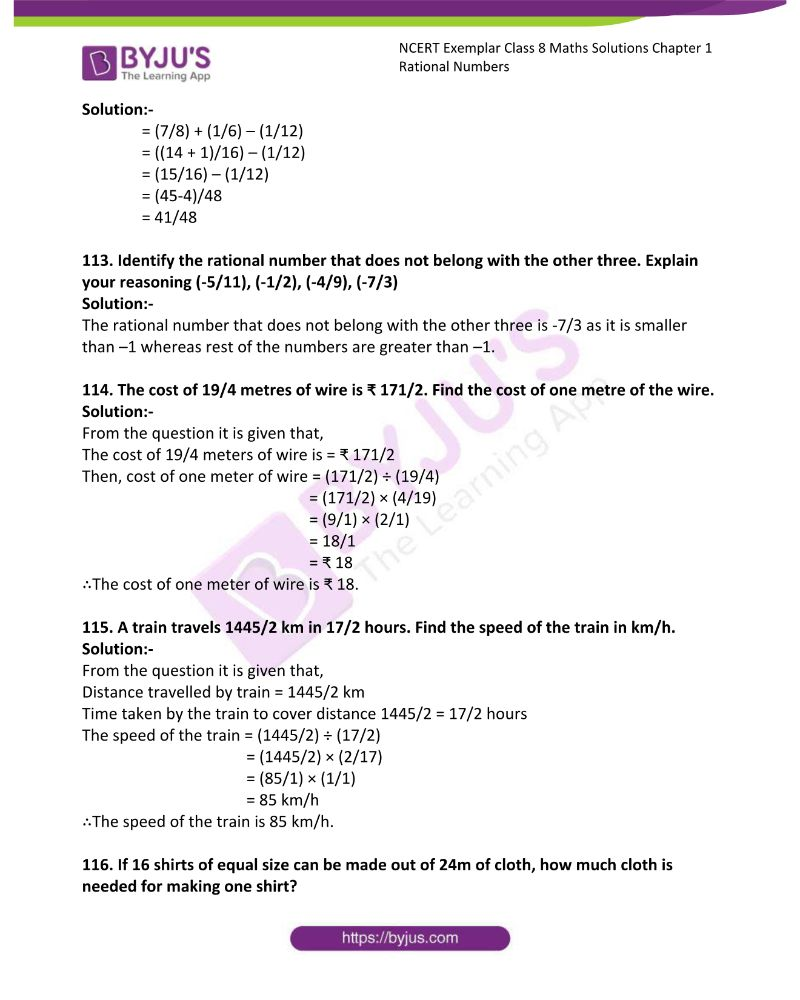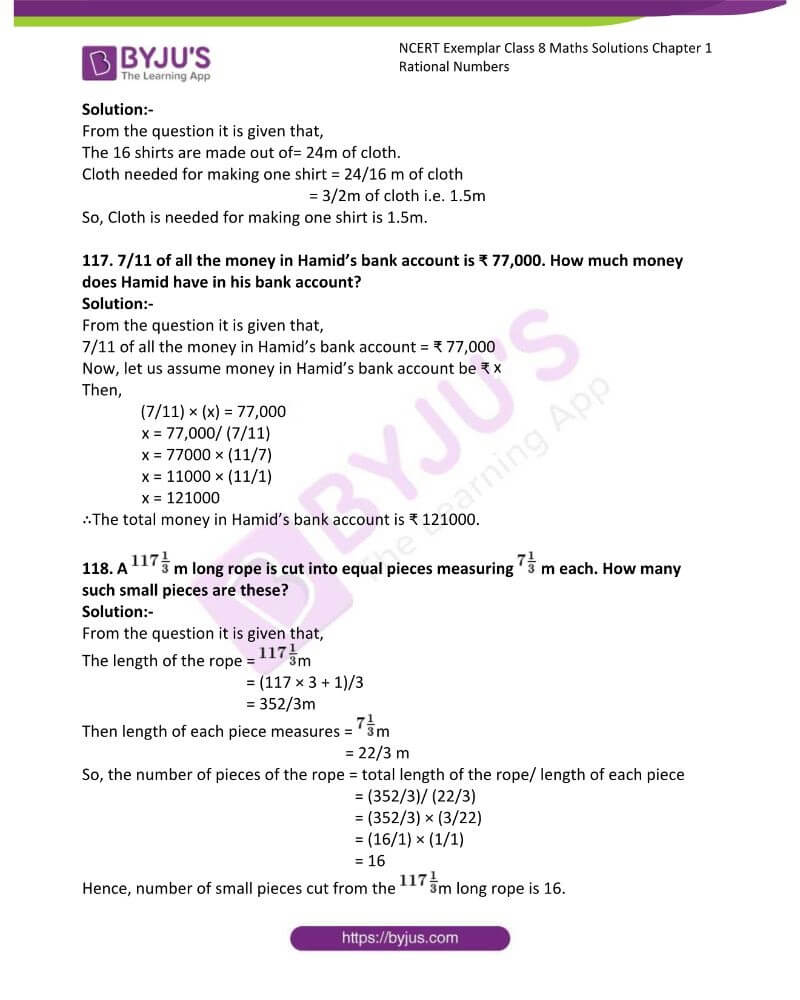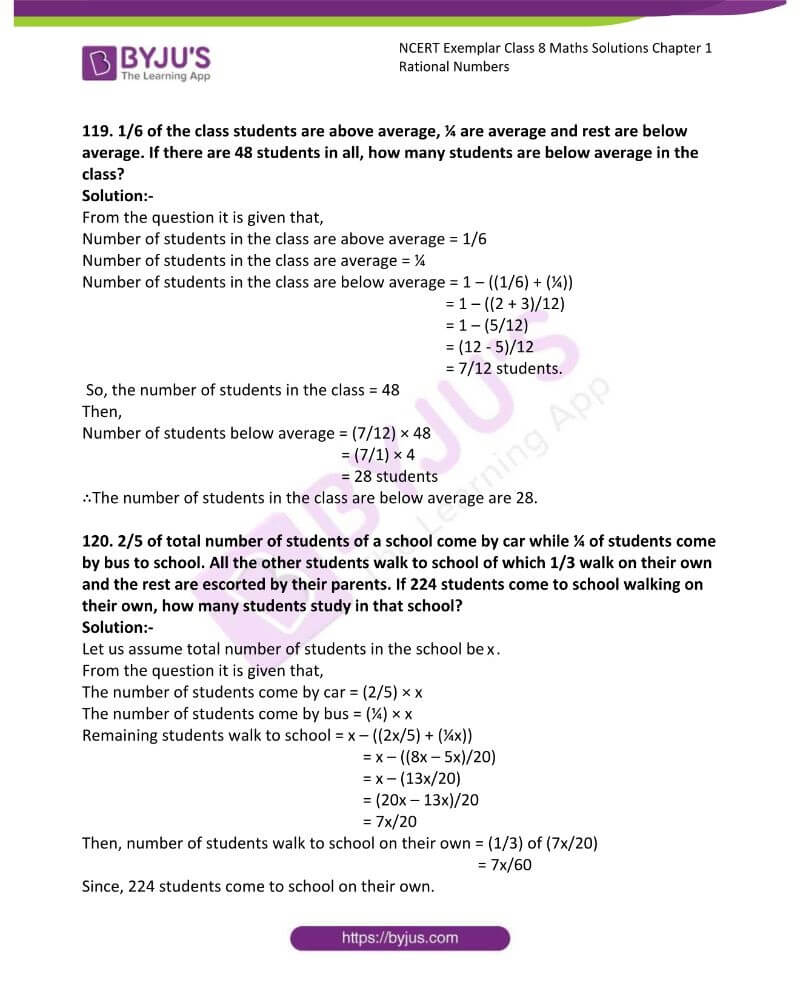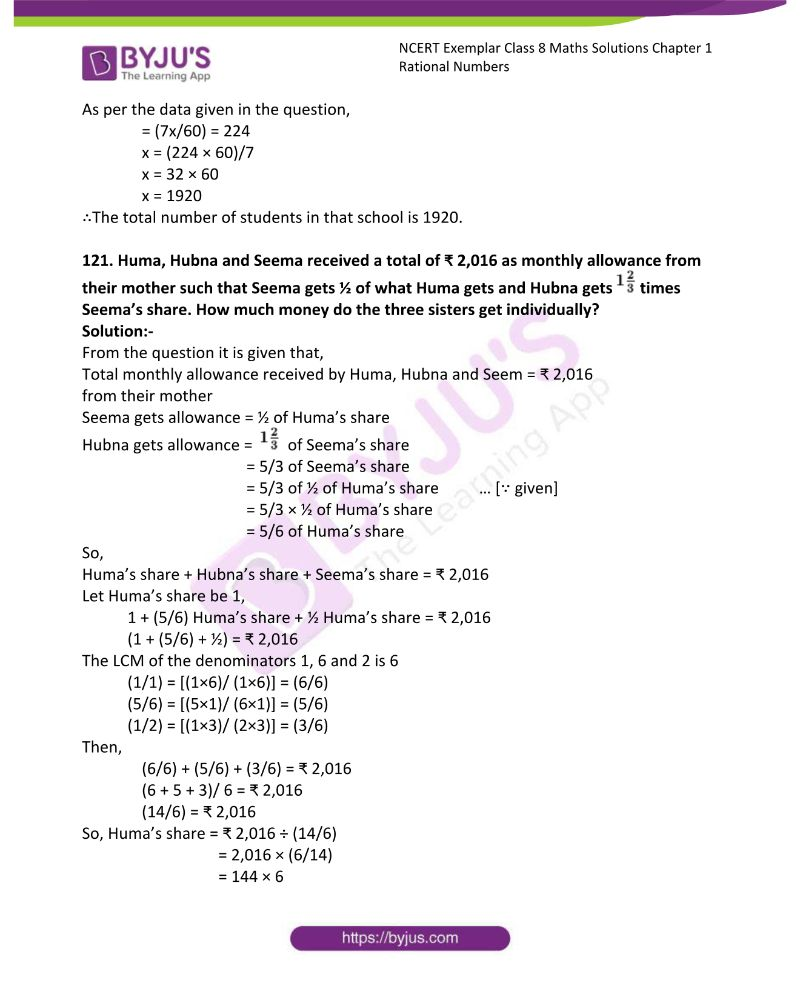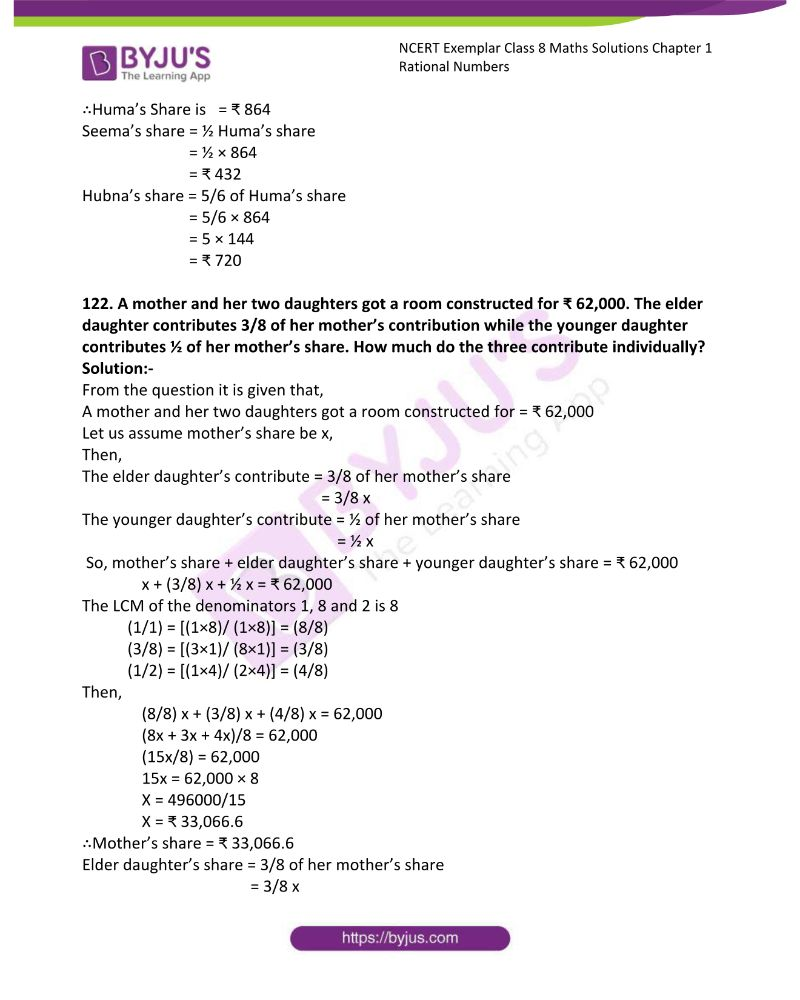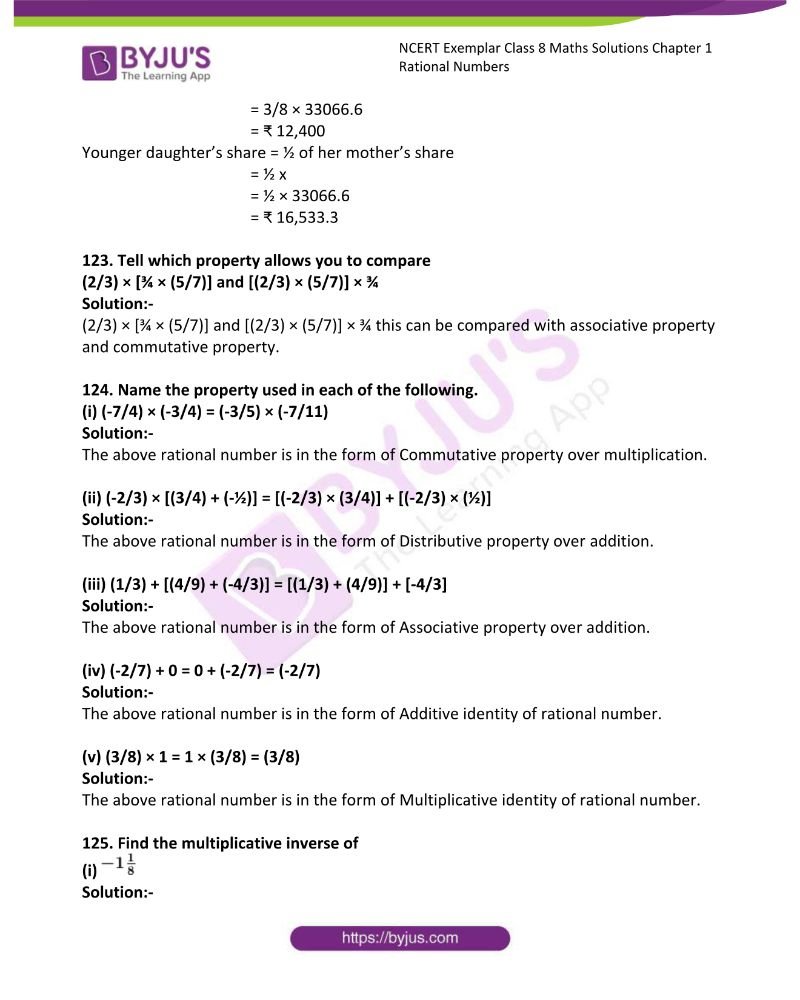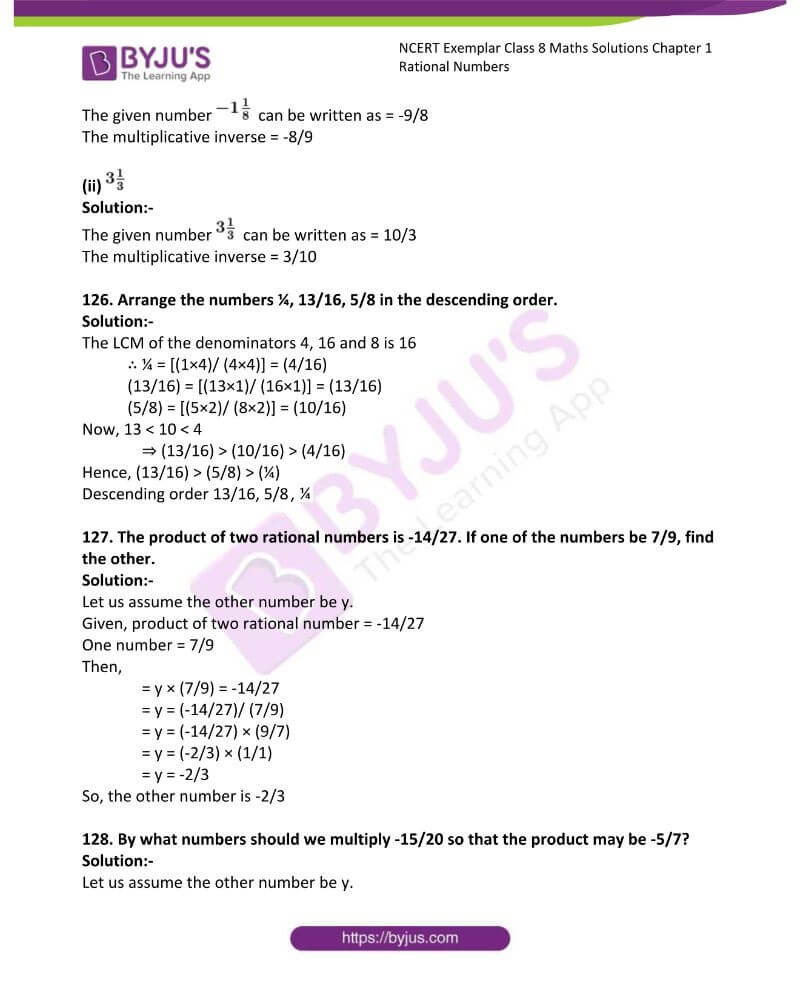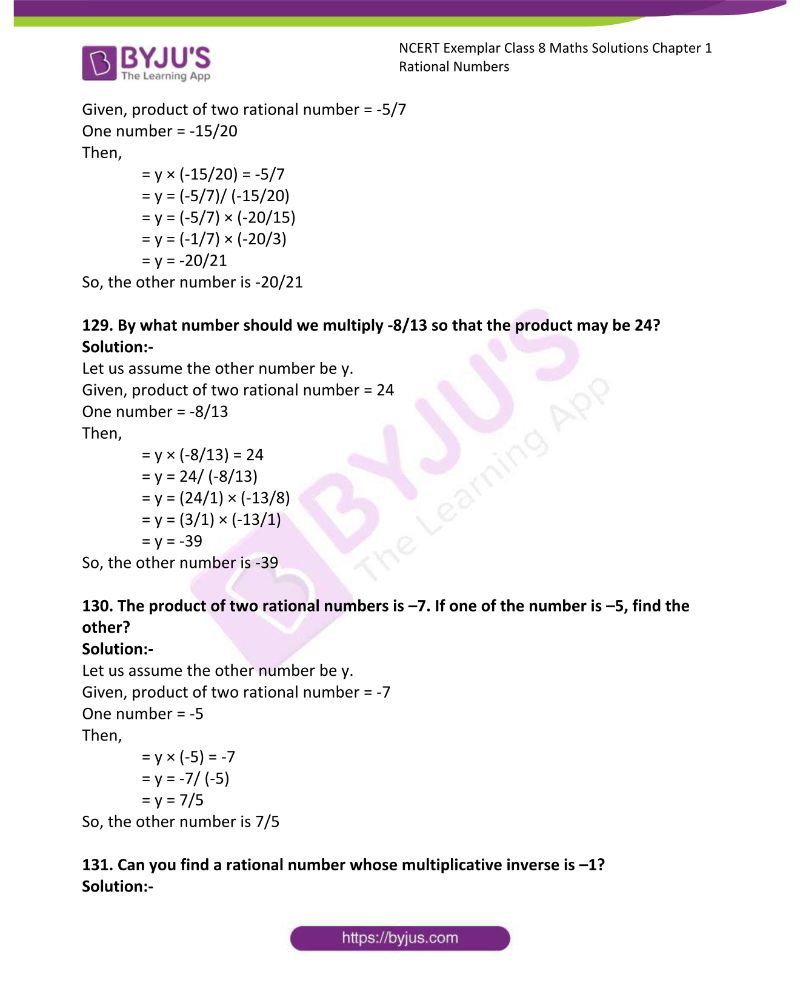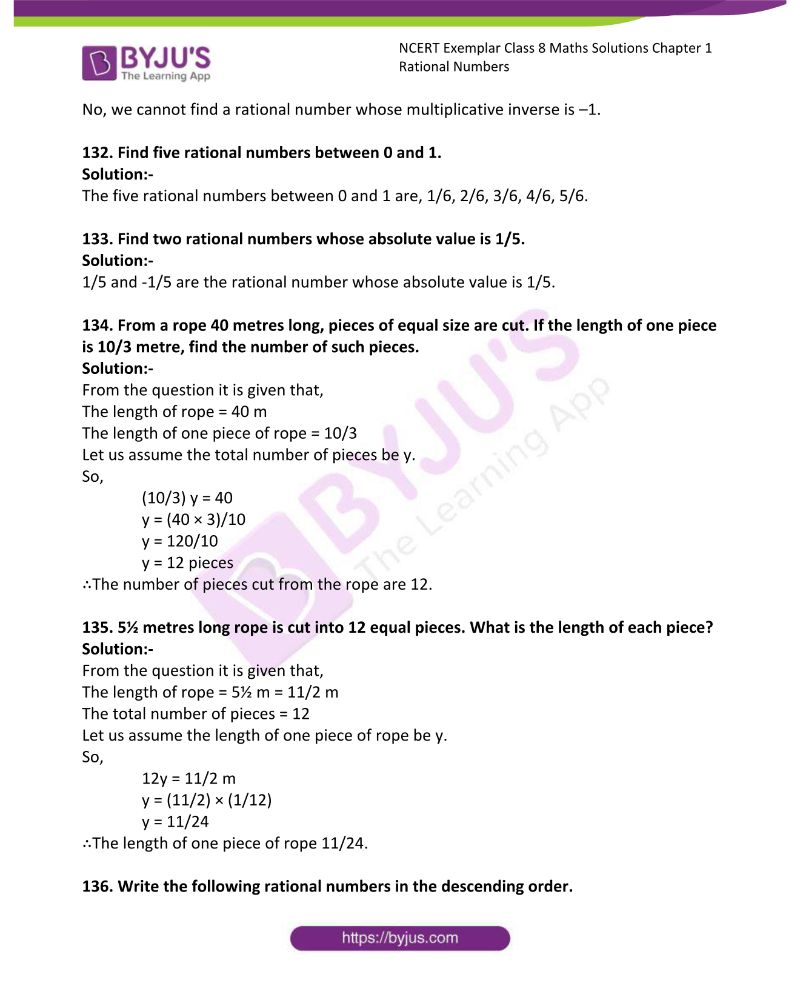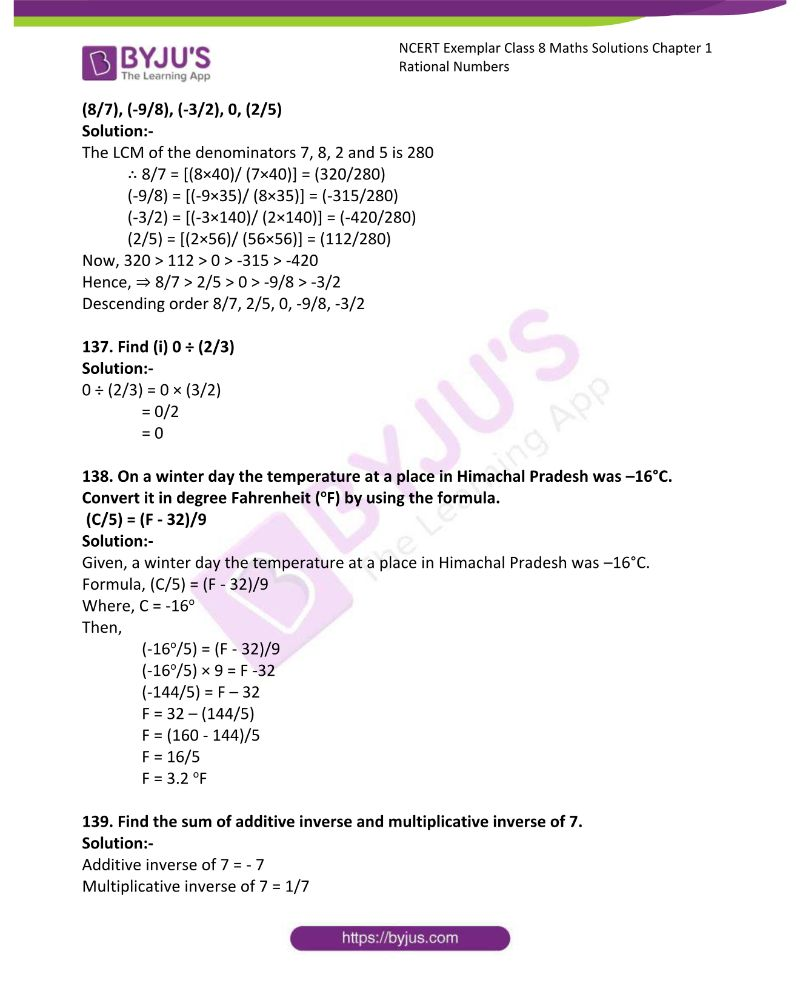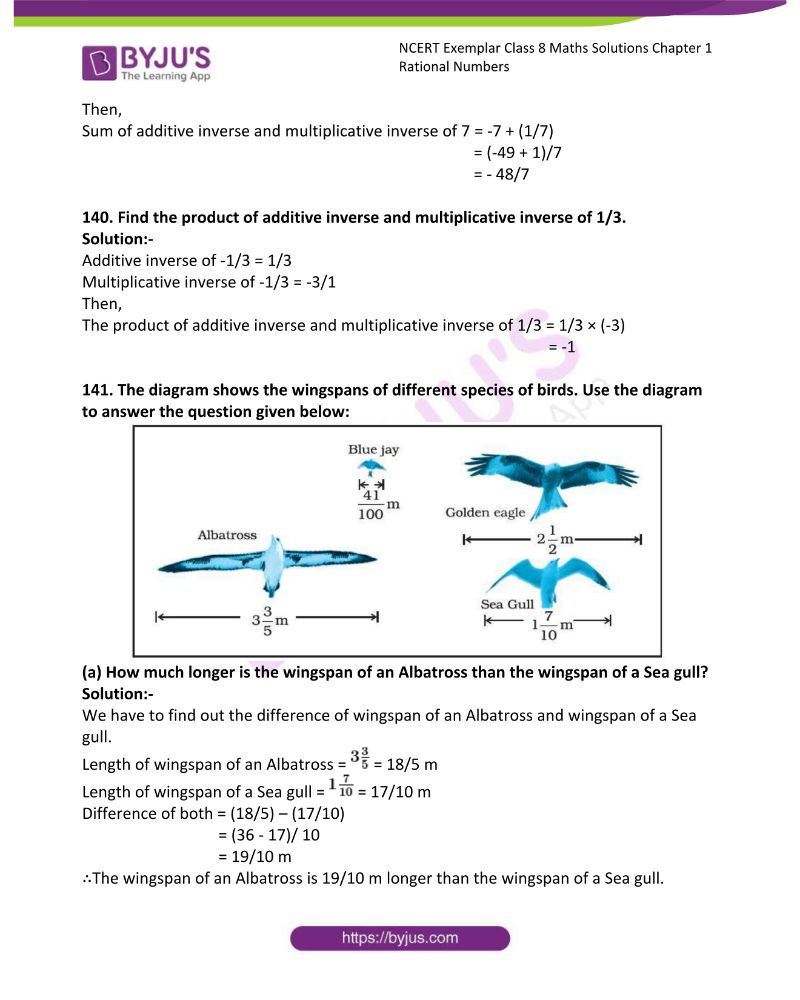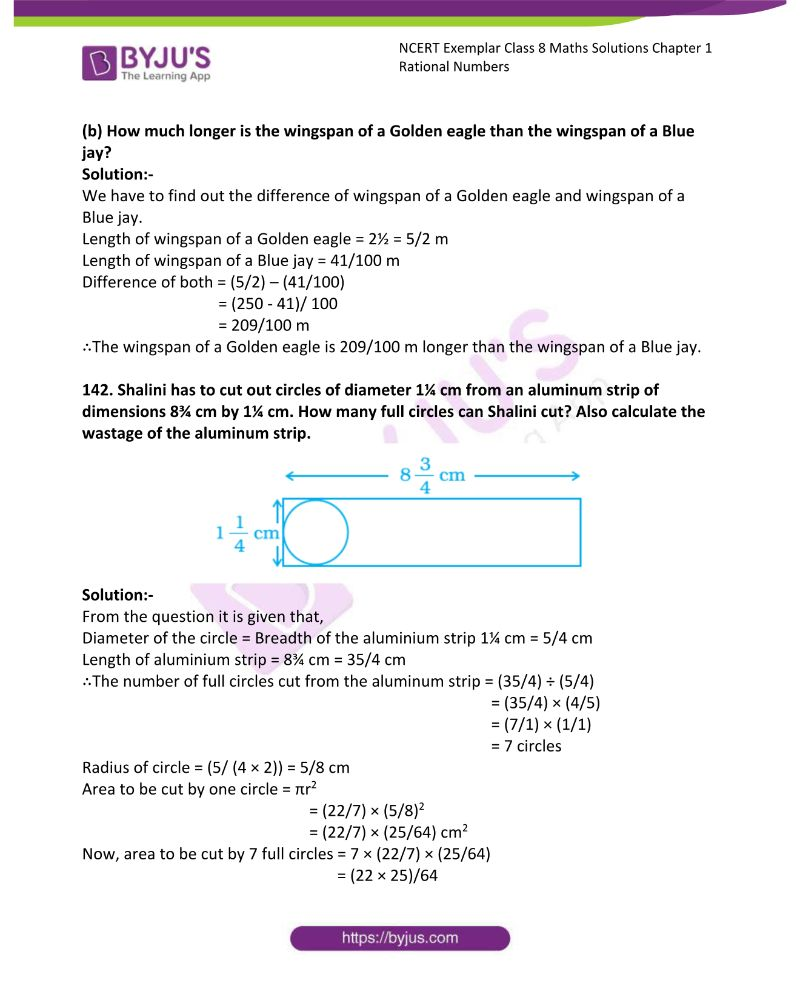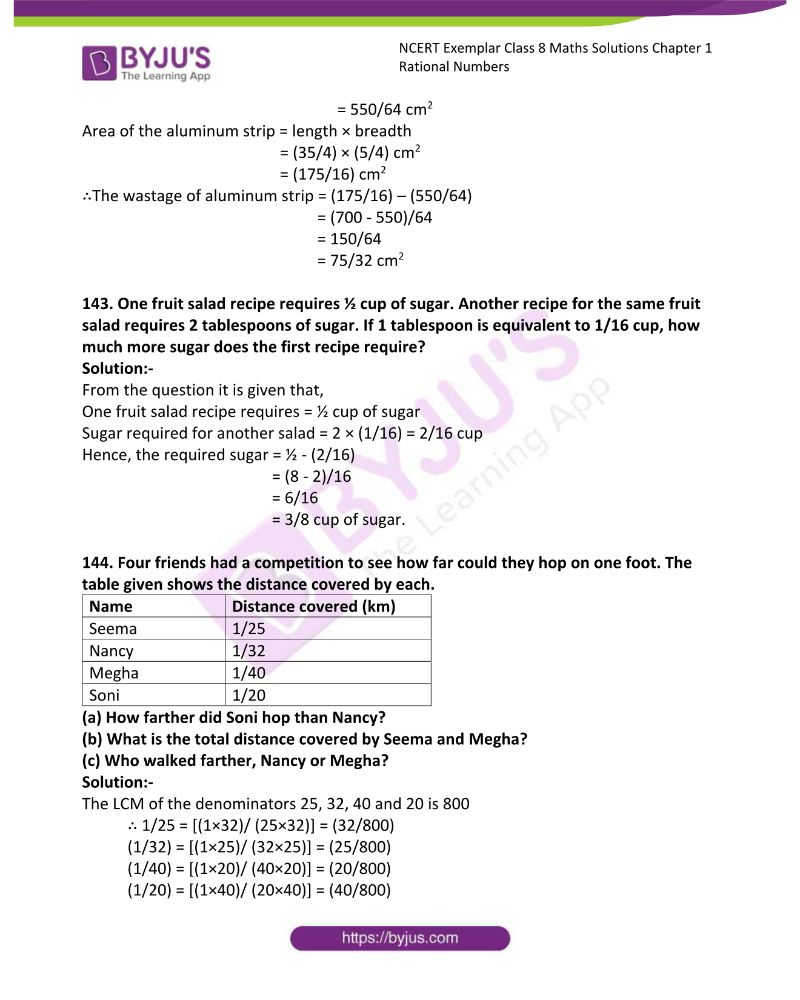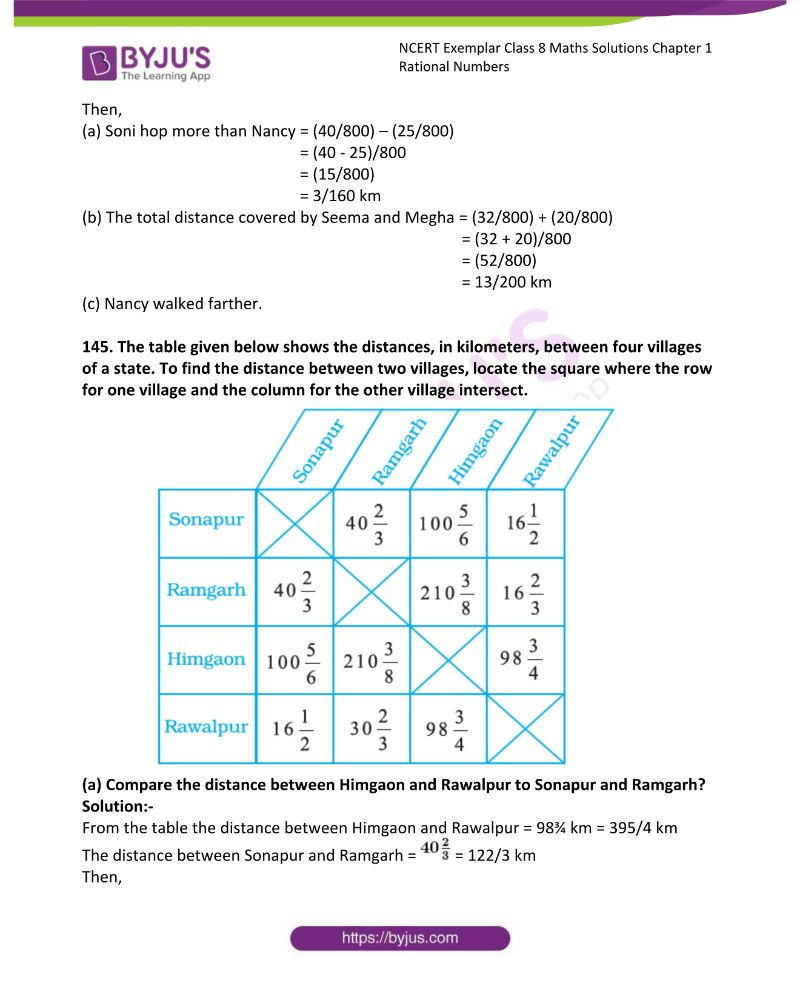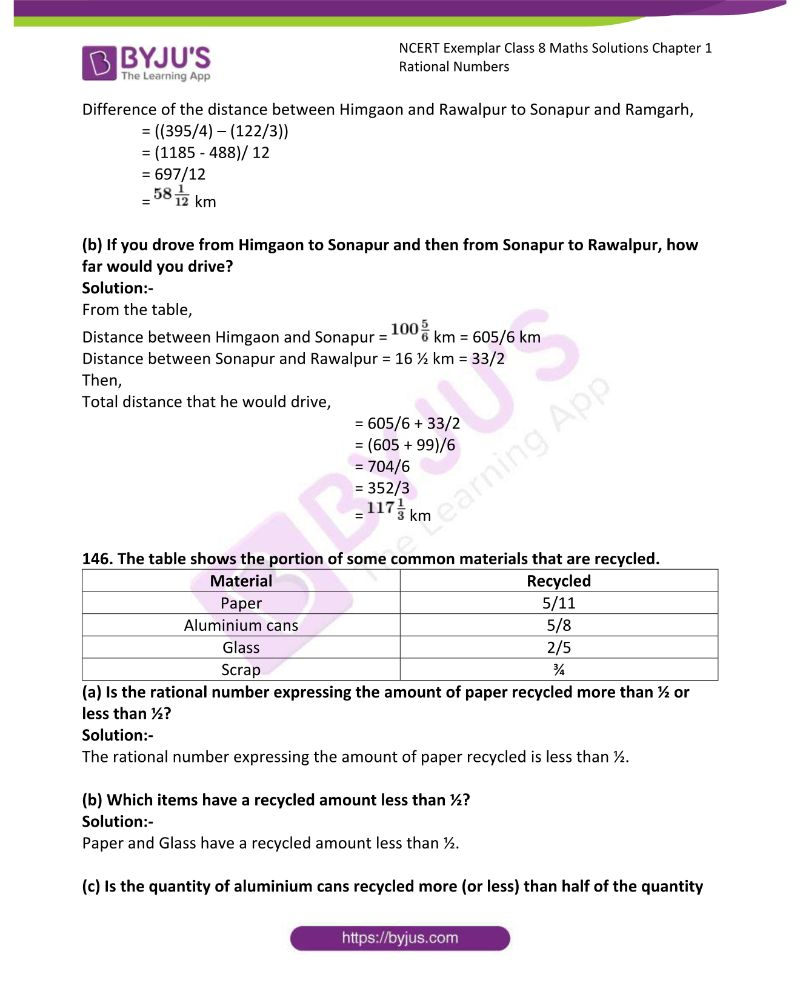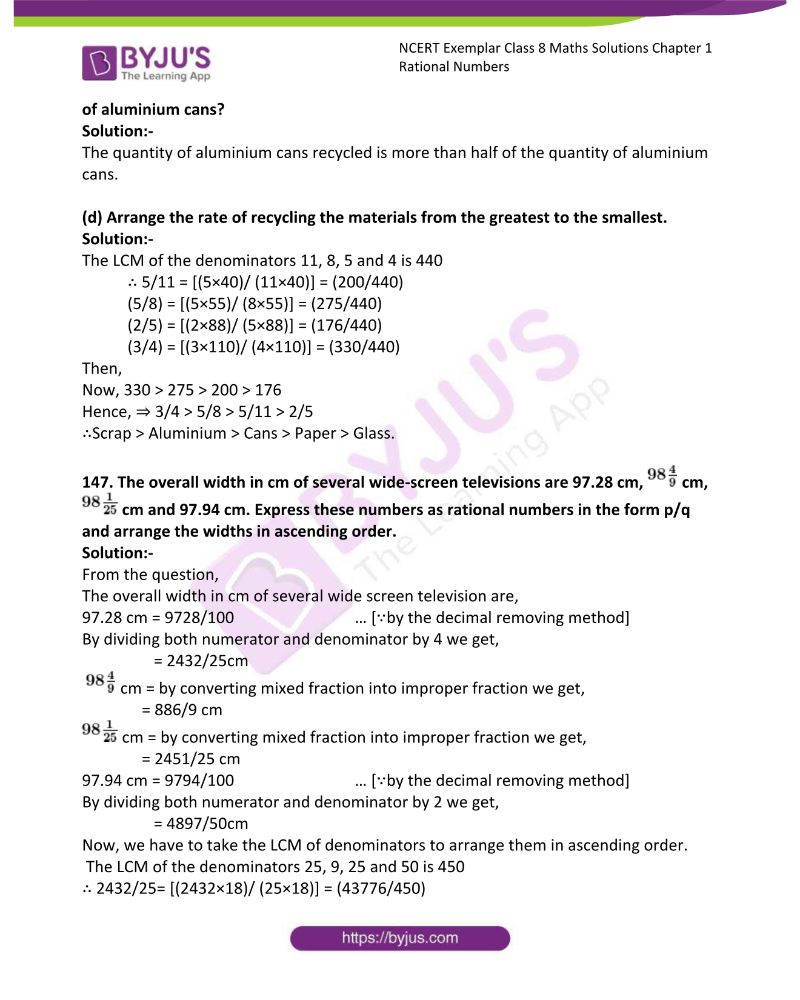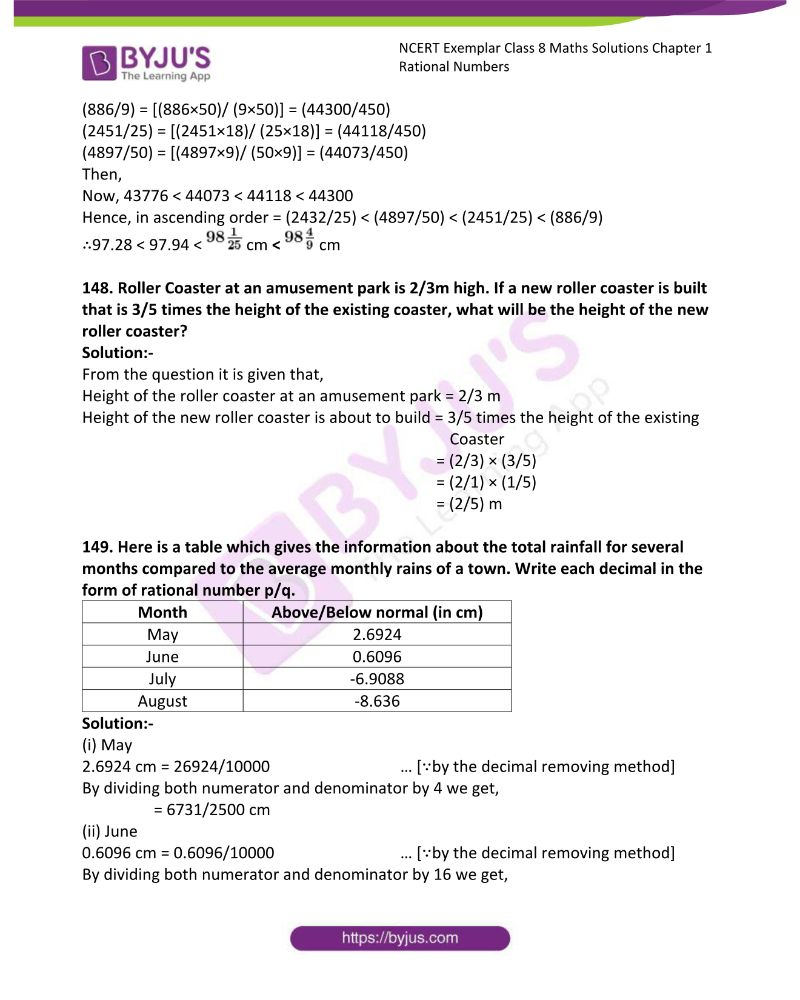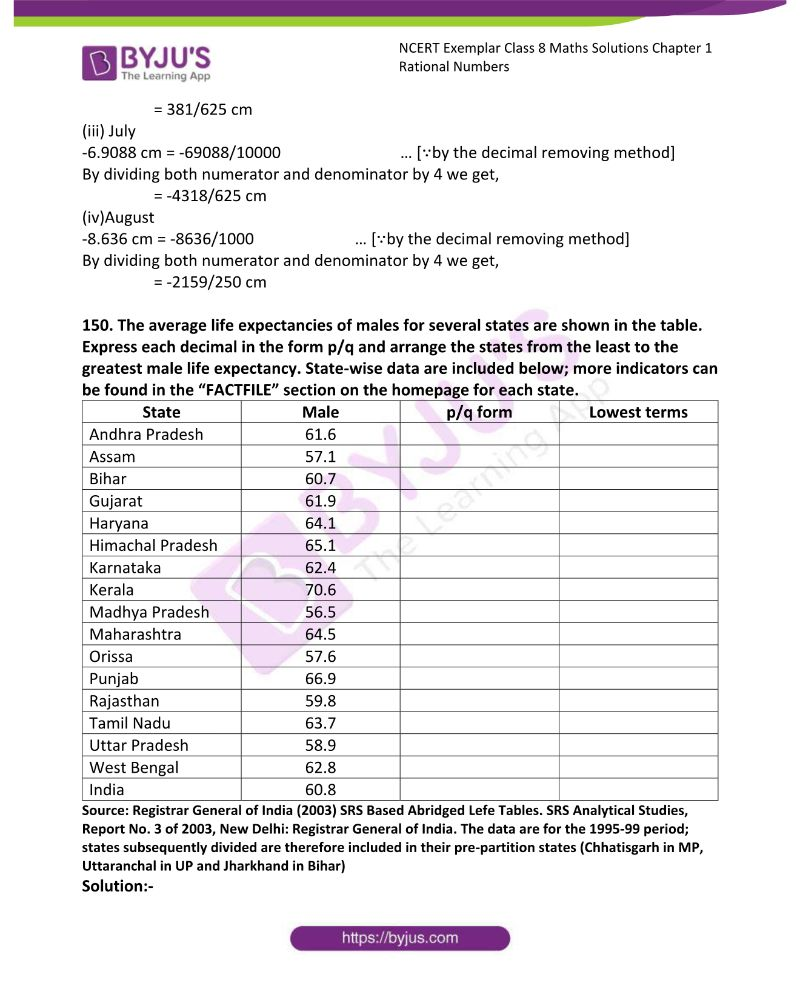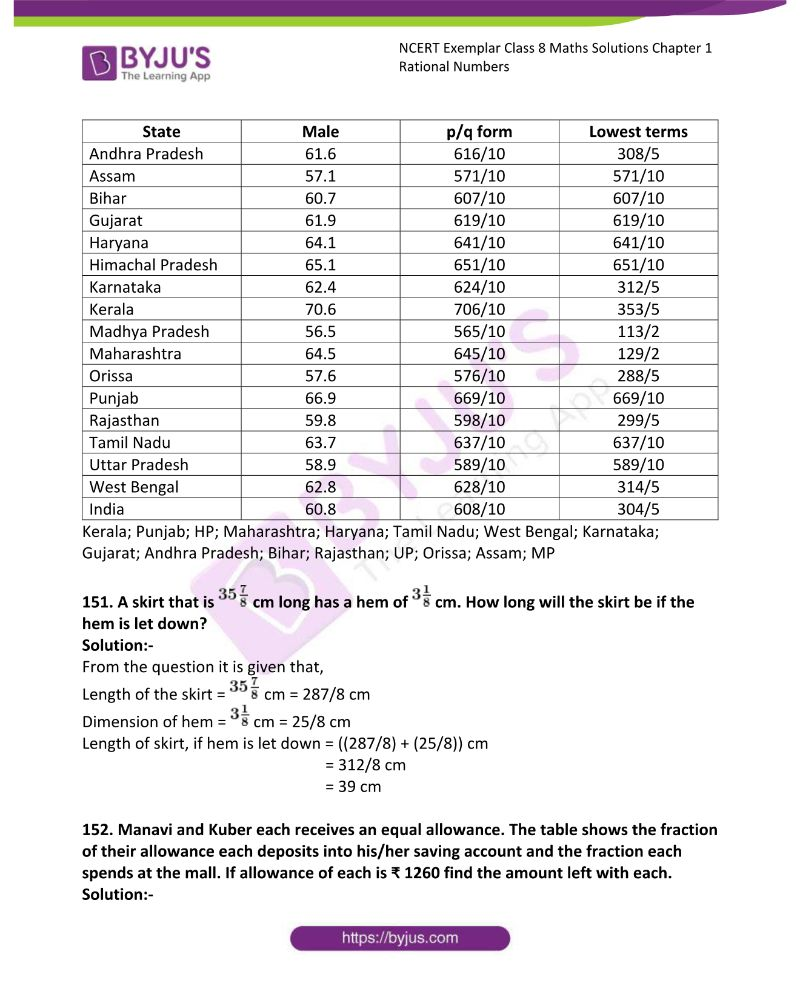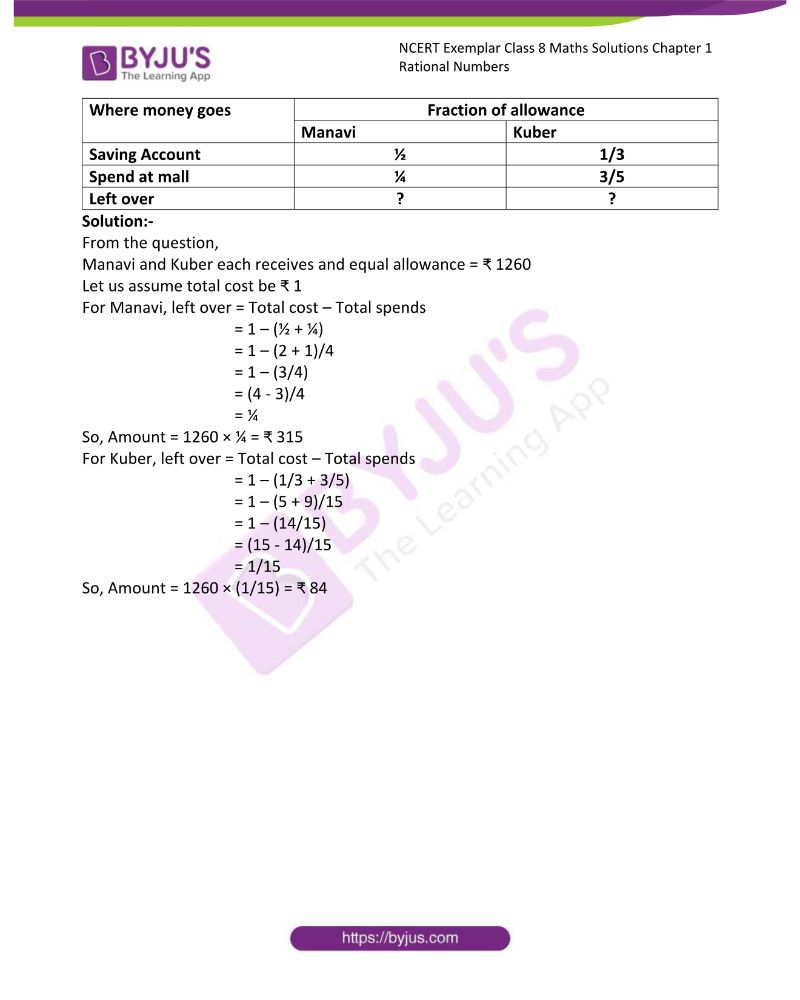### Access Answers to NCERT Exemplar Solutions Class 8 Maths Chapter 1 Rational Numbers

Exercise Page: 9

In question 1 to 25, there are four options out of which one is correct choose the correct answer.

1. A number which can be expressed as p/q where p and q are integers and q≠0 is

(a) natural number. (b) whole number.

(c) integer. (d) rational number

Solution:-

A number which can be expressed as p/q where p and q are integers and q≠0 is (d) rational number

2. A number of the form p/q is said to be a rational number if

(a) p and q are integers.

(b) p and q are integers and q ≠ 0

(c) p and q are integers and p ≠ 0

(d) p and q are integers and p ≠ 0 also q ≠ 0

Solution:-

A number of the form p/q is said to be a rational number if (b) p and q are integers and q ≠ 0

3. The numerical expression (3/8) + (-5/7) = (-19/56) shows that

(a) rational numbers are closed under addition.

(b) rational numbers are not closed under addition.

(c) rational numbers are closed under multiplication.

(d) addition of rational numbers is not commutative.

Solution:-

(a) rational numbers are closed under addition.

Because, (3/8) + (-5/7)

Take the LCM of the denominators of the given rational numbers.

LCM of 8 and 7 is 56

Express each of the given rational numbers with the above LCM as the common denominator.

Now,

(3/8)= [(3×7)/ (8×7)] = (21/56)

(-5/7)= [(-5×8)/ (7×8)] = (-40/56)

Then,

= (21/56) + (-40/56) … [∵ denominator is same in both the rational numbers]

= (21 – 40)/56

= (-19/56)

4. . Which of the following is not true?

(a) rational numbers are closed under addition.

(b) rational numbers are closed under subtraction.

(c) rational numbers are closed under multiplication.

(d) rational numbers are closed under division.

Solution:-

(d) rational numbers are closed under division.

Because, rational numbers are closed under the operations of addition, subtraction and multiplication.

5. (-3/8) + (1/7) = (1/7) + (-3/8) is an example to show that

(a) addition of rational numbers is commutative.

(b) rational numbers are closed under addition.

(c) addition of rational number is associative.

(d) rational numbers are distributive under addition.

Solution:-

(a) addition of rational numbers is commutative.

The arrangement of above rational numbers is in the form of Commutative law of addition [a + b=b + a]

6. Which of the following expressions shows that rational numbers are associative under multiplication.

(a) [(2/3) × ((-6/7) × (3/5))] = [((2/3) × (-6/7)) × (3/5)]

(b) [(2/3) × ((-6/7) × (3/5))] = [(2/3) × ((3/5) × (-6/7))]

(c) [(2/3) × ((-6/7) × (3/5))] = [((3/5) × (2/3)) × (-6/7)]

(d) [((2/3) × (-6/7)) × (3/5)] = [((-6/7) × (2/3)) × (3/5)]

Solution:-

(a) [(2/3) × ((-6/7) × (3/5))] = [((2/3) × (-6/7)) × (3/5)]

Because, the arrangement of above rational numbers is in the form of Associative law of Multiplication [a × (b ×c)] = [(a× b) × c]

7. Zero (0) is

(a) the identity for addition of rational numbers.

(b) the identity for subtraction of rational numbers.

(c) the identity for multiplication of rational numbers.

(d) the identity for division of rational numbers.

Solution:-

Zero (0) is (a) the identity for addition of rational numbers.

8. One (1) is

(a) the identity for addition of rational numbers.

(b) the identity for subtraction of rational numbers.

(c) the identity for multiplication of rational numbers.

(d) the identity for division of rational numbers.

Solution:-

One (1) is the identity for multiplication of rational numbers.

9. The additive inverse of -7/19 is

(a) -7/19 (b) 7/19 (c) 19/7 (d) -19/7

Solution:-

Additive inverse of (-7/19) is (b) (7/19)

The additive inverse of the rational number -a/b is a/b and vice-versa.

10. Multiplicative inverse of a negative rational number is

(a) a positive rational number.

(b) a negative rational number.

(c) 0

(d) 1

Solution:-

(b) a negative rational number.

(-1/3) is a rational number so its multiplicative inverse is (-3/1)

So that their multiplication will be,

= (-1/3) × (-3/1)

= – 1 × -1

= 1

11. If x + 0 = 0 + x = x, which is rational number, then 0 is called

(a) identity for addition of rational numbers.

(c) multiplicative inverse of x.

(d) reciprocal of x.

Solution:-

(a) identity for addition of rational numbers.

12. To get the product 1, we should multiply (8/21) by

(a) 8/21 (b) -8/21 (c) 21/8 (d) -21/8

Solution:-

(c) 21/8

Because,

= (8/21) × (21/8)

= (8 × 21) / (21 × 8)

= 168/168

= 1

13. – (-x) is same as

(a) –x (b) x (c) 1/x (d) -1/x

Solution:-

(b) x

We know that, (- × – = +)

14. The multiplicative inverse of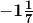is

(a) 8/7 (b) -8/7 (c) 7/8 (d) 7/-8

Solution:-

(d) 7/-8

== – 8/7

= 7/-8 [∵ reciprocal]

15. If x be any rational number then x + 0 is equal to

(a) x (b) 0 (c) –x (d) Not defined

Solution:-

(a) x

= x + 0 = x [∵ identity for addition of rational numbers]

16. The reciprocal of 1 is

(a) 1 (b) -1 (c) 0 (d) Not defined

Solution:-

(a) 1

Reciprocal of 1 = 1/1

= 1

17. The reciprocal of -1 is

(a) 1 (b) -1 (c) 0 (d) Not defined

Solution:-

(b) -1

Reciprocal of -1 = -1/1

= -1

18. The reciprocal of 0 is

(a) 1 (b) -1 (c) 0 (d) Not defined

Solution:-

(d) Not defined

Reciprocal of 0 = 1/0

= not defined

19. The reciprocal of any rational number p/q, where p and q are integers and q ≠ 0, is

(a) p/q (b) 1 (c) 0 (d) q/p

Solution:-

(d) q/p

The reciprocal of p/q = q/p

20. If y be the reciprocal of rational number x, then the reciprocal of y will be

(a) x (b) y (c) x/y (d) y/x

Solution:-

(a) x

If y be the reciprocal of rational number x, i.e. y = 1/x

x = 1/y

Then,

Reciprocal of y = x

21. The reciprocal of (-3/8) × (-7/13) is

(a) 104/21 (b) -104/21 (c) 21/104 (d) -21/104

Solution:-

(a) 104/21

= (-3 × -7) / (8 × 13)

= (21/104)

Reciprocal of 21/104 is 104/21

22. Which of the following is an example of distributive property of multiplication over addition for rational numbers.

(a) – (1/4) × {(2/3) + (-4/7)} = [-(1/4) × (2/3)] + [(-1/4) × (-4/7)]

(b) – (1/4) × {(2/3) + (-4/7)} = [(1/4) × (2/3)] – (-4/7)

(c) – (1/4) × {(2/3) + (-4/7)} = (2/3) + (-1/4) × (-4/7)

(d) – (1/4) × {(2/3) + (-4/7)} = {(2/3) + (-4/7)} – (1/4)

Solution:-

(a) – (1/4) × {(2/3) + (-4/7)} = [-(1/4) × (2/3)] + [(-1/4) × (-4/7)]

Because, we know the rule of distributive law, i.e. a × (b + c)] = [(a × b) + (a × c)

23. Between two given rational numbers, we can find

(a) one and only one rational number.

(b) only two rational numbers.

(c) only ten rational numbers.

(d) infinitely many rational numbers.

Solution:-

(d) infinitely many rational numbers.

24. (x + y)/2 is a rational number

(a) Between x and y

(b) Less than x and y both.

(c) Greater than x and y both.

(d) Less than x but greater than y

Solution:-

(a) Between x and y

Let us assume the value of x and y is 4 and 8 respectively

Then,

= (4 + 8)/ 2

= 12/2

= 6

Hence, the value 6 is lies between 4 and 8.

25. Which of the following statements is always true?

(a) (x – y)/2 is a rational number between x and y.

(b) (x + y)/2 is a rational number between x and y.

(c) (x × y)/2 is a rational number between x and y.

(d) (x ÷ y)/2 is a rational number between x and y.

Solution:-

(b) (x + y)/2 is a rational number between x and y

Let us assume the value of x and y is 6 and 9 respectively

Then,

= (6 + 9)/ 2

= 14/2

= 7

Hence, the value 7 is lies between 6 and 9.

In questions 26 to 47, fill in the blanks to make the statements true.

26. The equivalent of 5/7, whose numerator is 45 is .

Solution:-

Form the question it is given that equivalent of 5/7 = 45/denominator

To get 45 in the numerator multiply both numerator and denominator by 9

Then,

= (5 × 9)/ (7 × 9)

= 45/63

So, the equivalent of 5/7, whose numerator is 45 is (45/63)

27. The equivalent rational number of 7/9, whose denominator is 45 is .

Solution:-

Form the question it is given that equivalent of 7/9 = Numerator/45

To get 45 in the denominator multiply both numerator and denominator by 5

Then,

= (7 × 5)/ (9 × 5)

= 35/45

So, the equivalent rational number of 7/9, whose denominator is 45 is (35/45)

28. Between the numbers (15/20) and (35/40), the greater number is .

Solution:-

The LCM of the denominators 20 and 40 is 40

∴ (15/20) = [(15×2)/ (20×2)] = (30/40)

and (35/40) = [(35×1)/ (40×1)] = (35/40)

Now, 30 < 35

⇒ (30/40) < (35/40)

Hence, (15/20) < (35/40)

∴ 35/40 is greater.

So, between the numbers (15/20) and (35/40), the greater number is (35/40).

29. The reciprocal of a positive rational number is .

Solution:-

The reciprocal of a positive rational number is positive rational number.

Let us take positive rational number 2/3

The reciprocal of this positive rational number is 3/2 (positive rational number)

30. The reciprocal of a negative rational number is .

Solution:-

The reciprocal of a negative rational number is negative rational number.

Let us take negative rational number -3/4

The reciprocal of a negative rational number is 4/-3 = -4/3

31. Zero has reciprocal.

Solution:-

Zero has no reciprocal.

The reciprocal of 0 = 1/0

= Undefined

32. The numbers and are their own reciprocal.

Solution:-

The numbers 1 and -1 are their own reciprocal.

Reciprocal of 1 = 1/1 = 1

Reciprocal of -1 = 1/-1 = -1

33. If y be the reciprocal of x, then the reciprocal of y2 in terms of x will be .

Solution:-

If y be the reciprocal of x, then the reciprocal of y2 in terms of x will be x2.

From the question, (1/x) = y

Then,

Reciprocal of y2 = 1/y2

Substitute (1/x) in the place of y,

= 1/ (1/x)2

= x2/1

= x2

34. The reciprocal of (2/5) × (-4/9) is .

Solution:-

= (2 × -4)/ (5 × 9)

= -8/45

Reciprocal = -45/8

Hence, the reciprocal of (2/5) × (-4/5) is -45/8.

35. (213 × 657)-1 = 213-1 × .

Solution:-

Let us assume the missing number be x

Then,

= 1 / (213 × 657) = (1/213) × (x)

X = 213/ (213/657)

X = 1/657

X = 657-1

So, (213 × 657)-1 = 213-1 × 657-1

36. The negative of 1 is .

Solution:-

The negative of 1 is -1.

37. For rational numbers (a/b), (c/d) and (e/f) we have (a/b) × ((c/d) + (e/f)) = +

Solution:-

For rational numbers (a/b), (c/d) and (e/f) we have (a/b) × ((c/d) + (e/f)) = ((a/b) × (c/d)) + ((a/b) × (e/f))

38. -5/7 is than -3.

Solution:-

-5/7 is more than -3.

39. There are rational numbers between any two rational numbers.

Solution:-

There are infinitely many rational numbers between any two rational numbers.

40. The rational numbers 1/3 and -1/3 are on the sides of zero on the number line.

Solution:-

The rational numbers 1/3 and -1/3 are on the opposite sides of zero on the number line.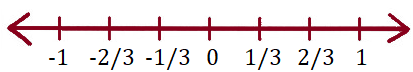41. The negative of a negative rational number is always a ________ rational number.

Solution:-

The negative of a negative rational number is always a positive rational number.

Example, let – a be a negative rational number.

Then, negative of a negative rational number = – (-a) = a positive rational number.

42. Rational numbers can be added or multiplied in any .

Solution:-

Rational numbers can be added or multiplied in any order.

43. The reciprocal of -5/7 is .

Solution:-

The reciprocal of -5/7 is -7/5.

44. The multiplicative inverse of 4/3 is .

Solution:-

The multiplicative inverse of 4/3 is ¾.

45. The rational number 10.11 in the from p/q is .

Solution:-

The rational number 10.11 in the from p/q is 1011/100.

46. (1/5) × [(2/7) + (3/8)] = [(1/5) × (2/7)] + .

Solution:-

(1/5) × [(2/7) + (3/8)] = [(1/5) × (2/7)] + [(1/5) × (3/8)]

∵ From the rule of distributive law of multiplication [a × (b + c) = (a × b) + (a × c)]

47. The two rational numbers lying between –2 and –5 with denominator as 1 are _________ and _________.

Solution:-

The two rational numbers lying between –2 and –5 with denominator as 1 are -3 and -4.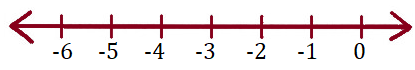In each of the following, state whether the statements are true (T) or false (F).

48. If x/y is a rational number, then y is always a whole number.

Solution:-

False.

If x/y is a rational numbers, then y is not equal to 0.

But 0 is a whole number.

49. If p/q is a rational number, then p cannot be equal to zero.

Solution:-

False.

If p/q is a rational number, p can be equal to zero (0) or any integer.

50. If r/s is a rational number, then s cannot be equal to zero.

Solution:-

True.

51. 5/6 lies between 2/3 and 1.

Solution:-

True.

Express each of the given rational numbers with 6 as the common denominator.

Now,

(2/3)= [(2×3)/ (2×2)] = (4/6)

(1)= [(1×6)/ (1×6)] = (6/6)

Then,

= 4/6 < 5/6 < 6/6

= 2/3 < 5/6 < 1

So, 5/6 lies between 2/3 and 1.

52. 5/10 lies between ½ and 1.

Solution:-

False.

Express each of the given rational numbers with 10 as the common denominator.

Now,

½ = [(1×5)/ (2×5)] = (5/10)

(1)= [(1×10)/ (1×10)] = (10/10)

Then,

½ is equal to 5/10

So, 5/6 does not lies between ½ and 1.

53. -7/2 lies between -3 and -4.

Solution:-

True.

Express each of the given rational numbers with 2 as the common denominator.

Now,

-3/1 = [(-3×2)/ (1×2)] = (-6/2)

-4/1 = [(-4×2)/ (1×2)] = (-8/2)

Then,

-8/2 > -7/2 > -6/2

-4 > -7/2 > -3

So, -7/2 is lies between -3 and -4.

54. 9/6 lies between 1 and 2.

Solution:-

True.

Express each of the given rational numbers with 6 as the common denominator.

Now,

1/1 = [(1×6)/ (1×6)] = (6/6)

2/1 = [(2×6)/ (1×6)] = (12/6)

Then,

6/6 < 9/6 < 12/6

1 < 9/6 < 2

So, 9/6 is lies between 1 and 2.

55. If a ≠ 0, the multiplicative inverse of a/b is b/a.

Solution:-

True.

56. The multiplicative inverse of -3/5 is 5/3

Solution:-

False.

Because, the correct answer is reciprocal of a negative rational number is negative rational number. i.e. reciprocal of -3/5 is -5/3.

57. The additive inverse of ½ is -2.

Solution:-

False.

The additive inverse of ½ is -½.

58. If x/y is the additive inverse of c/d, then (x/y) + (c/d) = 0

Solution:-

True.

Let x/y = ½ and its additive inverse c/d = -1/2

Then, (x/y) + (c/d)

= ½ + (-½ )

= ½ – ½

= 0

59. For every rational number x, x + 1 = x.

Solution:-

False.

Let x = 3

Then, 3 + 1 = 4

3 ≠ 4

So, it is clear that x + 1 ≠ x

60. If x/y is the additive inverse of c/d, then, (x/y) – (c/d) = 0

Solution:-

False.

Let x/y = 2/3 and its additive inverse c/d = -2/3

Then, (x/y) – (c/d)

= (2/3) – (-2/3)

= (2/3) + (2/3)

= 4/3

61. The reciprocal of a non-zero rational number q/p is the rational number q/p.

Solution:-

False.

Reciprocal of non-zero rational number q/p is p/q.

62. If x + y = 0, then –y is known as the negative of x, where x and y are rational numbers.

Solution:-

False.

If x and y are rational numbers, then y is known as the negative of x

63. The negative of the negative of any rational number is the number itself.

Solution:-

True.

Let y be a positive rational number.

Then,

The negative of the negative of y is = – (- y)

= y

64. The negative of 0 does not exist.

Solution:-

True.

65. The negative of 1 is 1 itself.

Solution:-

False.

The negative of 1 = -1

66. For all rational numbers x and y, x – y = y – x.

Solution:-

False.

Let x = 2, y = 3

Then,

LHS = x – y

= 2 – 3

= -1

RHS = y – x

= 3 – 2

= 1

By comparing LHS and RHS

-1 ≠ 1

LHS ≠ RHS

67. For all rational numbers x and y, (x) × (y) = (y) × (x)

Solution:-

True.

Let x = 2, y = 3

Then,

LHS = 2 × 3

= 6

RHS = 3 × 2

= 6

By comparing LHS and RHS

6 = 6

LHS = RHS

68. For every rational number x, x × 0 = x.

Solution:-

False.

Let x = 2

Then,

For every rational number x

(x) × (0) = 0

2 × 0 = 0

69. For every rational numbers x, y and z, x + (y × z) = (x + y) × (x + z).

Solution:-

False.

For every rational numbers a, b and c, [a × (b + c) = (a × b) + (a × c)]

70. For all rational numbers a, b and c, a (b + c) = ab + bc.

Solution:-

False.

Because, for every rational numbers a, b and c, [a × (b + c) = (a × b) + (a × c)]

71. 1 is the only number which is its own reciprocal.

Solution:-

False.

Because, the reciprocal of -1 is -1 and reciprocal of 1 is 1.

72. –1 is not the reciprocal of any rational number.

Solution:-

False.

The reciprocal of-1 is -1.

73. For any rational number x, x + (–1) = –x.

Solution:-

False.

The correct form is for any rational number x, (x) × (-1) = – x.

74. For rational numbers x and y, if x < y then x – y is a positive rational number.

Solution:-

False.

Because, for rational numbers x and y, if x < y then x – y is a negative rational number.

Example, let x = 2 and y = 3

Then,

= X – y

= 2 – 3

= -1

75. If x and y are negative rational numbers, then so is x + y.

Solution:-

True.

For example,

Let x = – 1/3 and y = -2/3

Then,

= x + y

= (-1/3) + (-2/3)

= -1/3 – 2/3

= -3/3

= – 1

76. Between any two rational numbers there are exactly ten rational numbers.

Solution:-

False.

Between any two rational numbers there are infinite rational numbers.

77. Rational numbers are closed under addition and multiplication but not under subtraction.

Solution:-

False.

Rational numbers are closed under addition, subtraction and multiplication.

78. Subtraction of rational number is commutative.

Solution:-

False.

Subtraction of rational number is not commutative.

Let x and y are any two rational number,

Then, x – y ≠ y – x

79. -¾ is smaller than -2.

Solution:-

False.

Express each of the given rational numbers with 4 as the common denominator.

Now,

-¾ = [(-3×1)/ (4×1)] = (-3/4)

-2/1 = [(-2×4)/ (1×4)] = (-8/4)

Then,

-3/4 > -8/4

Hence,

-¾ > -2

So, -¾ is greater than -2.

80. 0 is a rational number.

Solution:-

True.

Because, 0/1 is a rational number.

81. All positive rational numbers lie between 0 and 1000.

Solution:-

False.

There are infinite positive rational number on the right side of 0 on the number line.

82. The population of India in 2004 – 05 is a rational number.

Solution:-

True.

The population of India can always be a whole number. Hence, it is also a rational number.

83. There are countless rational numbers between 5/6 and 8/9.

Solution:-

True.

84. The reciprocal of x-1 is 1/x.

Solution:-

False.

X-1 = 1/x

Then, reciprocal of 1/x = x/1 = x

85. The rational number 57/23 lies to the left of zero on the number line.

Solution:-

False.

The given rational number is positive so it is lies to the right side of 0 on the number line.

86. The rational number 7/-4 is lies to the right side zero on the number line.

Solution:-

False.

The given rational number is negative so it is lies to the left side of 0 on the number line.

87. The rational number -8/-3 lies neither to the right nor to the left of zero on the number line.

Solution:-

False.

-8/-3 is written as 8/3 it is a positive rational number. So it is lies to the right side of 0 on the number line.

88. The rational numbers ½ and –1 are on the opposite sides of zero on the number line.

Solution:-

True.

½ is positive rational number so it is lies to the right side of 0 on the number line.

-1 is negative rational number so it is lies to the left side of 0 on the number line.

89. Every fraction is a rational number.

Solution:-

True.

Because rational numbers can be expressed in the p/q form and fraction is also a part of whole which can be expressed in the form of p/q.

90. Every integer is a rational number.

Solution:-

True.

In integer denominator remain 1. So, every integer is a rational number.

91. The rational numbers can be represented on the number line.

Solution:-

True.

92. The negative of a negative rational number is a positive rational number.

Solution:-

True.

Example, let us take -½ is a negative rational number.

Then negative of negative rational number = – (-½)

= ½ (positive rational number)

93. If x and y are two rational numbers such that x > y, then x – y is always a positive rational number.

Solution:-

True.

Let x = 4, y = 2

Then,

= x – y

= 4 – 2

= 2

94. 0 is the smallest rational number

Solution:-

False.

Negative rational number below 0 is infinite. So, the smallest rational number does not exist.

95. Every whole number is an integer.

Solution:-

True.

Every whole number is an integer but, every integer is not whole number.

96. Every whole number is a rational number.

Solution:-

True.

97. 0 is whole number but it is not a rational number.

Solution:-

False.

0 is whole number and also a rational number.

98. The rational numbers ½ and -5/2 are on the opposite sides of 0 on the number line.

Solution:-

True.

½ is positive rational number so it is lies to the right side of 0 on the number line.

-5/2 is negative rational number so it is lies to the left side of 0 on the number line.

99. Rational numbers can be added (or multiplied) in any order

(-4/5) × (-6/5) = (-6/5) × (-4/5)

Solution:-

True.

The arrangements of given rational number is as per the commutative law under multiplication. i.e. a × b = b × c

100. Solve the following: Select the rational numbers from the list which are also the integers.

9/4, 8/4, 7/4, 6/4, 9/3, 8/3, 7/3, 6/3, 5/2, 4/2, 3/1, 3/2, 1/1, 0/1, -1/1, -2/1, -3/2, -4/2, -5/2, -6/2

Solution:-

The rational number from the given list which also the integers are,

8/4 = 2, 9/3 = 3, 6/3 = 2, 4/2 = 2, 3/1 = 3, 1/1 = 1, 0/1 = 0, -1/1 = -1, -2/1 = -2, -4/2 = -2, -6/2 = -3

101. Select those which can be written as a rational number with denominator 4 in their lowest form:

(7/8), (64/16), (36/-12), (-16/17), (5/-4), (140/28)

Solution:-

Rational number with denominator 4 in their lowest form are,

64/16 = 16/4, 36/-12 = 12/-4, 5/-4, 140/28 =20/4

102. Using suitable rearrangement and find the sum:

(a) (4/7) + (-4/9) + (3/7) + (-13/9)

Solution:-

First rearrange the rational numbers and add the numbers with same denominator.

= (4/7) + (3/7) – (4/9) – (13/9)

= ((4 + 3)/7) – ((4 + 13)/9)

= (7/7) – (17/9)

= 1 – (17/9)

= (9 – 17)/9

= -8/9

(b) -5 + (7/10) + (3/7) + (-3) + (5/45) + (-4/5)

Solution:-

= -5 + (-3) + (7/10) + (-4/5) + (3/7) + (5/14)

= 8 + [(7-8)/10] + [(6 + 5)/14]

= – 8 – (1/10) + (11/14)

LCM of 1, 10 and 14 is 70

= (-560 – 7 + 55)/70

= -512/70

= – 256/35

103. Verify – (-x) = x for

(i) x = 3/5

Solution:-

– x = -3/5

– (-x) = – (-3/5)

X = 3/5

(ii) x = -7/9

Solution:-

– x = – (-7/9)

-x = 7/9

– (-x) = – (7/9)

X = – 7/9

(iii) x = 13/-15

Solution:-

– x = – (-13/15)

-x = 13/15

– (-x) = – (13/15)

X = -13/15

104. Give one example each to show that the rational numbers are closed under addition, subtraction and multiplication. Are rational numbers closed under division? Give two examples in support of your answer.

Solution:-

Rational numbers are closed under addition:-

Example:- 5/4 + 1/2

The LCM of the denominators 4 and 2 is 4

∴ (5/4) = [(5×1)/ (4×1)] = (5/4)

and (1/2) = [(1×2)/ (4×2)] = (1/4)

Then,

= 5/4 + ¼

= (5 + 1)/ 4

= 6/4

= 3/2 is a rational number

Rational numbers are closed under subtraction:-

Example:- 5/4 – 1/2

The LCM of the denominators 4 and 2 is 4

∴ (5/4) = [(5×1)/ (4×1)] = (5/4)

and (1/2) = [(1×2)/ (4×2)] = (1/4)

Then,

= 5/4 – ¼

= (5 – 1)/ 4

= 4/4

= 1 is a rational number

Rational numbers are closed under addition:-

Example:- 5/4 × 1/2

= 5/8 is a rational number.

For any rational number x, x ÷ 0 is not defined,

Hence not all rational numbers are closed under division. We can say that except zero, all rational numbers are closed under division.

Example, ¾ ÷ 4/5

= ¾ × 5/4

= 15/16 is a rational number.

105. Verify the property x + y = y + x of rational numbers by taking

(a) x = ½, y = ½

Solution:-

In the question is given to verify the property = x + y = y + x

Where, x = ½, y = ½

Then, ½ + ½ = ½ + ½

LHS = ½ + ½

= (1 + 1)/2

= 2/2

= 1

RHS = ½ + ½

= (1 + 1)/2

= 2/2

= 1

By comparing LHS and RHS

LHS = RHS

∴ 1 = 1

Hence x + y = y + x

(b) x = -2/3, y = -5/6

Solution:-

In the question is given to verify the property = x + y = y + x

Where, x = -2/3, y = -5/6

Then, -2/3 + (-5/6) = -5/6 + (-2/3)

LHS = -2/3 + (-5/6)

= -2/3 – 5/6

The LCM of the denominators 3 and 6 is 6

(-2/3) = [(-2×2)/ (3×2)] = (-4/6)

and (-5/6) = [(-5×1)/ (6×1)] = (-5/6)

Then,

= – 4/6 – 5/6

= (- 4 – 5)/ 6

= – 9/6

RHS = -5/6 + (-2/3)

= -5/6 – 2/3

The LCM of the denominators 6 and 3 is 6

(-5/6) = [(-5×1)/ (6×1)] = (-5/6)

and (-2/3) = [(-2×2)/ (3×2)] = (-4/6)

Then,

= – 5/6 – 4/6

= (- 5 – 4)/ 6

= – 9/6

By comparing LHS and RHS

LHS = RHS

∴ -9/6 = -9/6

Hence x + y = y + x

(c) x = -3/7, y = 20/21

Solution:-

In the question is given to verify the property = x + y = y + x

Where, x = -3/7, y = 20/21

Then, -3/7 + 20/21 = 20/21 + (-3/7)

LHS = -3/7 + 20/21

The LCM of the denominators 7 and 21 is 21

(-3/7) = [(-3×3)/ (7×3)] = (-9/21)

and (20/21) = [(20×1)/ (21×1)] = (20/21)

Then,

= – 9/21 + 20/21

= (- 9 + 20)/ 21

= 11/21

RHS = 20/21 + (-3/7)

The LCM of the denominators 21 and 7 is 21

(20/21) = [(20×1)/ (21×1)] = (20/21)

and (-3/7) = [(-3×3)/ (7×3)] = (-9/21)

Then,

= 20/21 – 9/21

= (20 – 9)/ 21

= 11/21

By comparing LHS and RHS

LHS = RHS

∴ 11/21 = 11/21

Hence x + y = y + x

(d) x = -2/5, y = – 9/10

Solution:-

In the question is given to verify the property = x + y = y + x

Where, x = -2/5, y = -9/10

Then, -2/5 + (-9/10) = -9/10 + (-2/5)

LHS = -2/5 + (-9/10)

= -2/5 – 9/10

The LCM of the denominators 5 and 10 is 10

(-2/5) = [(-2×2)/ (5×2)] = (-4/10)

and (-9/10) = [(-9×1)/ (10×1)] = (-9/10)

Then,

= – 4/10 – 9/10

= (- 4 – 9)/ 10

= – 13/10

RHS = -9/10 + (-2/5)

= -9/10 – 2/5

The LCM of the denominators 10 and 5 is 10

(-9/10) = [(-9×1)/ (10×1)] = (-9/10)

and (-2/5) = [(-2×2)/ (5×2)] = (-4/10)

Then,

= – 9/10 – 4/10

= (- 9 – 4)/ 10

= – 13/10

By comparing LHS and RHS

LHS = RHS

∴ -13/10 = -13/10

Hence x + y = y + x

106. Simplify each of the following by using suitable property. Also name the property.

(a) [(½) × (¼)] + [(½) × 6]

Solution:-

The arrangement of the given rational number is as per the rule of distributive law over addition.

Now take out ½ as common.

Then,

= ½ [¼ + 6]

= ½ [(1 + 24)/4]

= ½ [25/24]

= ½ × (25/24)

= 25/8

(b) [(1/5) × (2/15)] – [(1/5) × (2/5)]

Solution:-

The arrangement of the given rational number is as per the rule of distributive law over subtraction.

Now take out 1/5 as common.

Then,

= 1/5 [(2/15) – (2/5)]

The LCM of the denominators 15 and 5 is 15

(2/15) = [(2×1)/ (15×1)] = (2/15)

and (2/5) = [(2×3)/ (5×3)] = (6/15)

= 1/5 [(2 – 6)/15]

= 1/5 [-4/15]

= (1/5) × (-4/15)

= -4/75

(c) (-3/5) × {(3/7) + (-5/6)}

Solution:-

The arrangement of the given rational number is as per the rule of distributive law over addition.

= (-3/5) × {(3/7) + (-5/6)}

The LCM of the denominators 7 and 6 is 42

(3/7) = [(3×6)/ (7×6)] = (18/42)

and (-5/6) = [(-5×7)/ (6×7)] = (-35/42)

= -3/5 [(18 – 35)/42]

= -3/5 [-17/42]

= (-3/5) × (-17/42)

= 51/210 … [divide both denominator and numerator by 3]

= 17/30

107. Tell which property allows you to compute

(1/5) × [(5/6) × (7/9)] as [(1/5) × (5/6)] × (7/9)

Solution:-

The arrangement of the given rational number is as per the rule of Associative property for Multiplication.

108. Verify the property x × y = y × z of rational numbers by using

(a) x = 7 and y = ½

Solution:-

In the question is given to verify the property = x × y = y × x

Where, x = 7, y = ½

Then, 7 × ½ = ½ × 7

LHS = 7 × ½

= 7/2

RHS = ½ × 7

= 7/2

By comparing LHS and RHS

LHS = RHS

∴ 7/2 = 7/2

Hence x × y = y × x

(b) x = 2/3 and y = 9/4

Solution:-

In the question is given to verify the property = x × y = y × x

Where, x = 2/3, y = 9/4

Then, (2/3) × (9/4) = (9/4) × (2/3)

LHS = (2/3) × (9/4)

= (1/1) × (3/2)

= 3/2

RHS = (9/4) × (2/3)

= (3/2) × (1/1)

= 3/2

By comparing LHS and RHS

LHS = RHS

∴ 3/2 = 3/2

Hence x × y = y × x

(c) x = -5/7 and y = 14/15

Solution:-

In the question is given to verify the property = x × y = y × x

Where, x = -5/7, y = 14/15

Then, (-5/7) × (14/15) = (14/15) × (-5/7)

LHS = (-5/7) × (14/15)

= (-1/1) × (2/3)

= -2/3

RHS = (14/15) × (-5/7)

= (2/3) × (-1/1)

= -2/3

By comparing LHS and RHS

LHS = RHS

∴ -2/3 = -2/3

Hence x × y = y × x

(d) x = -3/8 and y = -4/9

Solution:-

In the question is given to verify the property = x × y = y × x

Where, x = -3/8, y = -4/9

Then, (-3/8) × (-4/9) = (-4/9) × (-3/8)

LHS = (-3/8) × (-4/9)

= (-1/2) × (-1/3)

= 1/6

RHS = (-4/9) × (-3/8)

= (-1/3) × (-1/2)

= 1/6

By comparing LHS and RHS

LHS = RHS

∴ 1/6 = 1/6

Hence x × y = y × x

109. Verify the property x × (y × z) = (x × y) × z of rational numbers by using

(a) x = 1, y = -½ and z = ¼

Solution:-

In the question is given to verify the property x × (y × z) = (x × y) × z

The arrangement of the given rational number is as per the rule of associative property for multiplication.

Then, 1 × (-½ × ¼) = (1 × -½) × ¼

LHS = 1 × (-½ × ¼)

= 1 × (-1/8)

= -1/8

RHS = (1 × -½) × ¼

= (-½) × ¼

= -1/8

By comparing LHS and RHS

LHS = RHS

∴ -1/8 = -1/8

Hence x × (y × z) = (x × y) × z

(b) x = 2/3, y = -3/7 and z = ½

Solution:-

In the question is given to verify the property x × (y × z) = (x × y) × z

The arrangement of the given rational number is as per the rule of associative property for multiplication.

Then, (2/3) × (-3/7 × ½) = ((2/3) × (-3/7)) × ½

LHS = (2/3) × (-3/7 × ½)

= (2/3) × (-3/14)

= -6/42

RHS = ((2/3) × (-3/7)) × ½

= (-6/21) × ½

= -6/42

By comparing LHS and RHS

LHS = RHS

∴ -6/42 = -6/42

Hence x × (y × z) = (x × y) × z

(c) x = -2/7, y = -5/6 and z = ¼

Solution:-

In the question is given to verify the property x × (y × z) = (x × y) × z

The arrangement of the given rational number is as per the rule of associative property for multiplication.

Then, (-2/7) × (-5/6 × ¼) = ((-2/7) × (-5/6)) × ¼

LHS = (-2/7) × (-5/6 × ¼)

= (-2/7) × (-5/24)

= 10/168

RHS = ((-2/7) × (-5/6)) × ¼

= (10/42) × ¼

= 10/168

By comparing LHS and RHS

LHS = RHS

∴ 10/168 = 10/168

Hence x × (y × z) = (x × y) × z

110. Verify the property x × (y + z) = x × y + x × z of rational numbers by taking.

(a) x = -½, y = ¾, z = ¼

Solution:-

In the question is given to verify the property x × (y + z) = x × y + x × z

The arrangement of the given rational number is as per the rule of distributive property of multiplication over addition.

Then, (-½) × (¾ + ¼) = (-½ × ¾) + (-½ × ¼)

LHS = (-½) × (¾ + ¼)

= (-½) × ((3 + 1)/4)

= -½ × (4/4)

= -½ × 1

= -½

RHS = (-½ × ¾) + (-½ × ¼)

= (-3/8) + (-1/8)

= (-3 – 1)/8

= -4/8

= -½

By comparing LHS and RHS

LHS = RHS

∴ -½ = -½

Hence x × (y + z) = x × y + x × z

(b) x = -½, y = 2/3, z = ¾

Solution:-

In the question is given to verify the property x × (y + z) = x × y + x × z

The arrangement of the given rational number is as per the rule of distributive property of multiplication over addition.

Then, (-½) × ((2/3) + ¾) = (-½ × (2/3)) + (-½ × ¾)

LHS = (-½) × ((2/3) + ¾)

= (-½) × ((8 + 9)/12)

= -½ × (17/12)

= -17/24

RHS = (-½ × (2/3)) + (-½ × ¾)

= (-1/3) + (-3/8)

= (-8 – 9)/24

= -17/24

By comparing LHS and RHS

LHS = RHS

∴ -17/24 = -17/24

Hence x × (y + z) = x × y + x × z

(c) x = -2/3, y = -4/6, z = -7/9

Solution:-

In the question is given to verify the property x × (y + z) = x × y + x × z

The arrangement of the given rational number is as per the rule of distributive property of multiplication over addition.

Then, (-2/3) × ((-4/6) + (-7/9)) = ((-2/3) × (-4/6)) + ((-2/3) × (-7/9))

LHS = (-2/3) × ((-4/6) + (-7/9))

= (-2/3) × ((-12 – 14)/18)

= – (2/3) × (-26/18)

= – (1/3) × (-26/9)

= 26/27

RHS = ((-2/3) × (-4/6)) + ((-2/3) × (-7/9))

= ((-1/3) × (-4/3)) + ((-2/3) × (-7/9))

= (4/9) + (14/27)

= (12 + 14)/27

= 26/27

By comparing LHS and RHS

LHS = RHS

∴ 26/27 = 26/27

Hence x × (y + z) = x × y + x × z

(d) x = -1/5, y = 2/15, z = -3/10

Solution:-

In the question is given to verify the property x × (y + z) = x × y + x × z

The arrangement of the given rational number is as per the rule of distributive property of multiplication over addition.

Then, (-1/5) × ((2/15) + (-3/10)) = ((-1/5) × (2/15)) + ((-1/5) × (-3/10))

LHS = (-1/5) × ((2/15) + (-3/10))

= (-1/5) × ((4 – 9)/30)

= (-1/5) × (-5/30)

= (-1/1) × (-1/30)

= 1/30

RHS = ((-1/5) × (2/15)) + ((-1/5) × (-3/10))

= (-2/75) + (3/50)

= (-4 + 9)/150

= 5/150

= 1/30

By comparing LHS and RHS

LHS = RHS

∴ 1/30 = 1/30

Hence x × (y + z) = x × y + x × z

111. Use the distributivity of multiplication of rational numbers over addition to simplify.

(a) (3/5) × [(35/24) + (10/1)]

Solution:-

We know that the distributivity of multiplication of rational numbers over addition, a × (b + c) = a × b + a × c

Where, a =3/5, b =35/24, c = 10/1

Then, (3/5) × [(35/24) + (10/1)] = ((3/5) × (35/24)) + ((3/5) × (10/1))

= ((1/1) × (7/8)) + ((3/1) × (2/1))

= (7/8) + (6/1)

= (7 + 48)/8

= 55/8

=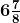(b) (-5/4) × [(8/5) + (16/15)]

Solution:-

We know that the distributivity of multiplication of rational numbers over addition, a × (b + c) = a × b + a × c

Where, a =-5/4, b =8/5, c = 16/15

Then, (-5/4) × [(8/5) + (16/15)] = ((-5/4) × (8/5)) + ((-5/4) × (16/15))

= ((-1/1) × (2/1)) + ((-1/1) × (4/3))

= (-2/1) + (-4/3)

= (-6 – 4)/3

= -10/3

=-3 1/3

(c) (2/7) × [(7/16) – (21/4)]

Solution:-

We know that the distributivity of multiplication of rational numbers over subtraction, a × (b – c) = a × b – a × c

Where, a = -2/7, b = 7/16, c = 21/4

Then, (2/7) × [(7/16) – (21/4)] = ((2/7) × (7/16)) – ((2/7) × (21/4))

= ((1/1) × (1/8)) – ((1/1) × (3/2))

= (1/8) – (3/2)

= (1 – 12)/8

= -11/8

(d) ¾ × [(8/9) – 40]

Solution:-

We know that the distributivity of multiplication of rational numbers over subtraction, a × (b – c) = a × b – a × c

Where, a = -2/7, b = 7/16, c = 21/4

Then, (¾) × [(8/9) – (40)] = ((¾) × (8/9)) – ((¾) × (40))

= ((1/1) × (2/3)) – ((3/1) × (10))

= (2/3) – (30)

= (2 – 90)/3

= -88/3

112. Simplify

(a) (32/5) + (23/11) × (22/15)

Solution:-

= (32/5) + (23/1) × (2/15)

= (32/5) + (46/15)

= (96 + 46)/15

= 142/15

(b) (3/7) × (28/15) ÷ (14/5)

Solution:-

= (3/7) × (28/15) ÷ (14/5)

= (1/1) × (4/5) ÷ (14/5)

= (4/5) ÷ (14/5)

= (4/5) × (5/14)

= (2/1) × (1/7)

= 2/7

(c) (3/7) + (-2/21) × (-5/6)

Solution:-

= (3/7) – (2/21) × (-5/6)

= (3/7) – (1/21) × (-5/3)

= (3/7) – (-5/63)

= (3/7) + (5/63)

= (27 + 5)/63

= 32/63

(d) (7/8) + (1/6) – (1/12)

Solution:-

= (7/8) + (1/6) – (1/12)

= ((14 + 1)/16) – (1/12)

= (15/16) – (1/12)

= (45-4)/48

= 41/48

113. Identify the rational number that does not belong with the other three. Explain your reasoning (-5/11), (-1/2), (-4/9), (-7/3)

Solution:-

The rational number that does not belong with the other three is -7/3 as it is smaller than –1 whereas rest of the numbers are greater than –1.

114. The cost of 19/4 metres of wire is ₹ 171/2. Find the cost of one metre of the wire.

Solution:-

From the question it is given that,

The cost of 19/4 meters of wire is = ₹ 171/2

Then, cost of one meter of wire = (171/2) ÷ (19/4)

= (171/2) × (4/19)

= (9/1) × (2/1)

= 18/1

= ₹ 18

∴The cost of one meter of wire is ₹ 18.

115. A train travels 1445/2 km in 17/2 hours. Find the speed of the train in km/h.

Solution:-

From the question it is given that,

Distance travelled by train = 1445/2 km

Time taken by the train to cover distance 1445/2 = 17/2 hours

The speed of the train = (1445/2) ÷ (17/2)

= (1445/2) × (2/17)

= (85/1) × (1/1)

= 85 km/h

∴The speed of the train is 85 km/h.

116. If 16 shirts of equal size can be made out of 24m of cloth, how much cloth is needed for making one shirt?

Solution:-

From the question it is given that,

The 16 shirts are made out of= 24m of cloth.

Cloth needed for making one shirt = 24/16 m of cloth

= 3/2m of cloth i.e. 1.5m

So, Cloth is needed for making one shirt is 1.5m.

117. 7/11 of all the money in Hamid’s bank account is ₹ 77,000. How much money does Hamid have in his bank account?

Solution:-

From the question, it is given that

7/11 of all the money in Hamid’s bank account = ₹ 77,000

Now, let us assume money in Hamid’s bank account be ₹ x.

Then,

(7/11) × (x) = 77,000

x = 77,000/ (7/11)

x = 77000 × (11/7)

x = 11000 × (11/1)

x = 121000

∴The total money in Hamid’s bank account is ₹ 121000.

118. A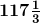m long rope is cut into equal pieces measuring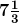m each. How many such small pieces are these?

Solution:-

From the question it is given that,

The length of the rope =m

= (117 × 3 + 1)/3

= 352/3m

Then length of each piece measures =m

= 22/3 m

So, the number of pieces of the rope = total length of the rope/ length of each piece

= (352/3)/ (22/3)

= (352/3) × (3/22)

= (16/1) × (1/1)

= 16

Hence, number of small pieces cut from them long rope is 16.

119. 1/6 of the class students are above average, ¼ are average and rest are below average. If there are 48 students in all, how many students are below average in the class?

Solution:-

From the question it is given that,

Number of students in the class are above average = 1/6

Number of students in the class are average = ¼

Number of students in the class are below average = 1 – ((1/6) + (¼))

= 1 – ((2 + 3)/12)

= 1 – (5/12)

= (12 – 5)/12

= 7/12 students.

So, the number of students in the class = 48

Then,

Number of students below average = (7/12) × 48

= (7/1) × 4

= 28 students

∴The number of students in the class are below average are 28.

120. 2/5 of total number of students of a school come by car while ¼ of students come by bus to school. All the other students walk to school of which 1/3 walk on their own and the rest are escorted by their parents. If 224 students come to school walking on their own, how many students study in that school?

Solution:-

Let us assume total number of students in the school be x.

From the question it is given that,

The number of students come by car = (2/5) × x

The number of students come by bus = (¼) × x

Remaining students walk to school = x – ((2x/5) + (¼x))

= x – ((8x – 5x)/20)

= x – (13x/20)

= (20x – 13x)/20

= 7x/20

Then, number of students walk to school on their own = (1/3) of (7x/20)

= 7x/60

Since, 224 students come to school on their own.

As per the data given in the question,

= (7x/60) = 224

x = (224 × 60)/7

x = 32 × 60

x = 1920

∴The total number of students in that school is 1920.

121. Huma, Hubna and Seema received a total of ₹ 2,016 as monthly allowance from their mother such that Seema gets ½ of what Huma gets and Hubna gets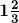times Seema’s share. How much money do the three sisters get individually?

Solution:-

From the question it is given that,

Total monthly allowance received by Huma, Hubna and Seem = ₹ 2,016

from their mother

Seema gets allowance = ½ of Huma’s share

Hubna gets allowance =of Seema’s share

= 5/3 of Seema’s share

= 5/3 of ½ of Huma’s share … [∵ given]

= 5/3 × ½ of Huma’s share

= 5/6 of Huma’s share

So,

Huma’s share + Hubna’s share + Seema’s share = ₹ 2,016

Let Huma’s share be 1,

1 + (5/6) Huma’s share + ½ Huma’s share = ₹ 2,016

(1 + (5/6) + ½) = ₹ 2,016

The LCM of the denominators 1, 6 and 2 is 6

(1/1) = [(1×6)/ (1×6)] = (6/6)

(5/6) = [(5×1)/ (6×1)] = (5/6)

(1/2) = [(1×3)/ (2×3)] = (3/6)

Then,

(6/6) + (5/6) + (3/6) = ₹ 2,016

(6 + 5 + 3)/ 6 = ₹ 2,016

(14/6) = ₹ 2,016

So, Huma’s share = ₹ 2,016 ÷ (14/6)

= 2,016 × (6/14)

= 144 × 6

∴Huma’s Share is = ₹ 864

Seema’s share = ½ Huma’s share

= ½ × 864

= ₹ 432

Hubna’s share = 5/6 of Huma’s share

= 5/6 × 864

= 5 × 144

= ₹ 720

122. A mother and her two daughters got a room constructed for ₹ 62,000. The elder daughter contributes 3/8 of her mother’s contribution while the younger daughter contributes ½ of her mother’s share. How much do the three contribute individually?

Solution:-

From the question it is given that,

A mother and her two daughters got a room constructed for = ₹ 62,000

Let us assume mother’s share be x,

Then,

The elder daughter’s contribute = 3/8 of her mother’s share

= 3/8 x

The younger daughter’s contribute = ½ of her mother’s share

= ½ x

So, mother’s share + elder daughter’s share + younger daughter’s share = ₹ 62,000

x + (3/8) x + ½ x = ₹ 62,000

The LCM of the denominators 1, 8 and 2 is 8

(1/1) = [(1×8)/ (1×8)] = (8/8)

(3/8) = [(3×1)/ (8×1)] = (3/8)

(1/2) = [(1×4)/ (2×4)] = (4/8)

Then,

(8/8) x + (3/8) x + (4/8) x = 62,000

(8x + 3x + 4x)/8 = 62,000

(15x/8) = 62,000

15x = 62,000 × 8

X = 496000/15

X = ₹ 33,066.6

∴Mother’s share = ₹ 33,066.6

Elder daughter’s share = 3/8 of her mother’s share

= 3/8 x

= 3/8 × 33066.6

= ₹ 12,400

Younger daughter’s share = ½ of her mother’s share

= ½ x

= ½ × 33066.6

= ₹ 16,533.3

123. Tell which property allows you to compare

(2/3) × [¾ × (5/7)] and [(2/3) × (5/7)] × ¾

Solution:-

(2/3) × [¾ × (5/7)] and [(2/3) × (5/7)] × ¾ this can be compared with associative property and commutative property.

124. Name the property used in each of the following.

(i) (-7/4) × (-3/4) = (-3/5) × (-7/11)

Solution:-

The above rational number is in the form of Commutative property over multiplication.

(ii) (-2/3) × [(3/4) + (-½)] = [(-2/3) × (3/4)] + [(-2/3) × (½)]

Solution:-

The above rational number is in the form of Distributive property over addition.

(iii) (1/3) + [(4/9) + (-4/3)] = [(1/3) + (4/9)] + [-4/3]

Solution:-

The above rational number is in the form of Associative property over addition.

(iv) (-2/7) + 0 = 0 + (-2/7) = (-2/7)

Solution:-

The above rational number is in the form of Additive identity of rational number.

(v) (3/8) × 1 = 1 × (3/8) = (3/8)

Solution:-

The above rational number is in the form of Multiplicative identity of rational number.

125. Find the multiplicative inverse of

(i)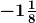Solution:-

The given numbercan be written as = -9/8

The multiplicative inverse = -8/9

(ii)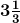Solution:-

The given numbercan be written as = 10/3

The multiplicative inverse = 3/10

126. Arrange the numbers ¼, 13/16, 5/8 in the descending order.

Solution:-

The LCM of the denominators 4, 16 and 8 is 16

∴ ¼ = [(1×4)/ (4×4)] = (4/16)

(13/16) = [(13×1)/ (16×1)] = (13/16)

(5/8) = [(5×2)/ (8×2)] = (10/16)

Now, 13 < 10 < 4

⇒ (13/16) > (10/16) > (4/16)

Hence, (13/16) > (5/8) > (¼)

Descending order 13/16, 5/8, 1/4

127. The product of two rational numbers is -14/27. If one of the numbers be 7/9, find the other.

Solution:-

Let us assume the other number be y.

Given, product of two rational number = -14/27

One number = 7/9

Then,

= y × (7/9) = -14/27

= y = (-14/27)/ (7/9)

= y = (-14/27) × (9/7)

= y = (-2/3) × (1/1)

= y = -2/3

So, the other number is -2/3

128. By what numbers should we multiply -15/20 so that the product may be -5/7?

Solution:-

Let us assume the other number be y.

Given, product of two rational number = -5/7

One number = -15/20

Then,

= y × (-15/20) = -5/7

= y = (-5/7)/ (-15/20)

= y = (-5/7) × (-20/15)

= y = (-1/7) × (-20/3)

= y = -20/21

So, the other number is -20/21

129. By what number should we multiply -8/13 so that the product may be 24?

Solution:-

Let us assume the other number be y.

Given, product of two rational number = 24

One number = -8/13

Then,

= y × (-8/13) = 24

= y = 24/ (-8/13)

= y = (24/1) × (-13/8)

= y = (3/1) × (-13/1)

= y = -39

So, the other number is -39

130. The product of two rational numbers is –7. If one of the number is –5, find the other?

Solution:-

Let us assume the other number be y.

Given, product of two rational number = -7

One number = -5

Then,

= y × (-5) = -7

= y = -7/ (-5)

= y = 7/5

So, the other number is 7/5

131. Can you find a rational number whose multiplicative inverse is –1?

Solution:-

No, we cannot find a rational number whose multiplicative inverse is –1.

132. Find five rational numbers between 0 and 1.

Solution:-

The five rational numbers between 0 and 1 are, 1/6, 2/6, 3/6, 4/6, 5/6.

133. Find two rational numbers whose absolute value is 1/5.

Solution:-

1/5 and -1/5 are the rational number whose absolute value is 1/5.

134. From a rope 40 metres long, pieces of equal size are cut. If the length of one piece is 10/3 metre, find the number of such pieces.

Solution:-

From the question it is given that,

The length of rope = 40 m

The length of one piece of rope = 10/3

Let us assume the total number of pieces be y.

So,

(10/3) y = 40

y = (40 × 3)/10

y = 120/10

y = 12 pieces

∴The number of pieces cut from the rope are 12.

135. 5½ metres long rope is cut into 12 equal pieces. What is the length of each piece?

Solution:-

From the question it is given that,

The length of rope = 5½ m = 11/2 m

The total number of pieces = 12

Let us assume the length of one piece of rope be y.

So,

12y = 11/2 m

y = (11/2) × (1/12)

y = 11/24

∴The length of one piece of rope 11/24.

136. Write the following rational numbers in the descending order.

(8/7), (-9/8), (-3/2), 0, (2/5)

Solution:-

The LCM of the denominators 7, 8, 2 and 5 is 280

∴ 8/7 = [(8×40)/ (7×40)] = (320/280)

(-9/8) = [(-9×35)/ (8×35)] = (-315/280)

(-3/2) = [(-3×140)/ (2×140)] = (-420/280)

(2/5) = [(2×56)/ (56×56)] = (112/280)

Now, 320 > 112 > 0 > -315 > -420

Hence, ⇒ 8/7 > 2/5 > 0 > -9/8 > -3/2

Descending order 8/7, 2/5, 0, -9/8, -3/2

137. Find (i) 0 ÷ (2/3)

Solution:-

0 ÷ (2/3) = 0 × (3/2)

= 0/2

= 0

138. On a winter day the temperature at a place in Himachal Pradesh was –16°C. Convert it in degree Fahrenheit (oF) by using the formula.

(C/5) = (F – 32)/9

Solution:-

Given, a winter day the temperature at a place in Himachal Pradesh was –16°C.

Formula, (C/5) = (F – 32)/9

Where, C = -16o

Then,

(-16o/5) = (F – 32)/9

(-16o/5) × 9 = F -32

(-144/5) = F – 32

F = 32 – (144/5)

F = (160 – 144)/5

F = 16/5

F = 3.2 oF

139. Find the sum of additive inverse and multiplicative inverse of 7.

Solution:-

Additive inverse of 7 = – 7

Multiplicative inverse of 7 = 1/7

Then,

Sum of additive inverse and multiplicative inverse of 7 = -7 + (1/7)

= (-49 + 1)/7

= – 48/7

140. Find the product of additive inverse and multiplicative inverse of 1/3.

Solution:-

Additive inverse of -1/3 = 1/3

Multiplicative inverse of -1/3 = -3/1

Then,

The product of additive inverse and multiplicative inverse of 1/3 = 1/3 × (-3)

= -1

141. The diagram shows the wingspans of different species of birds. Use the diagram to answer the question given below: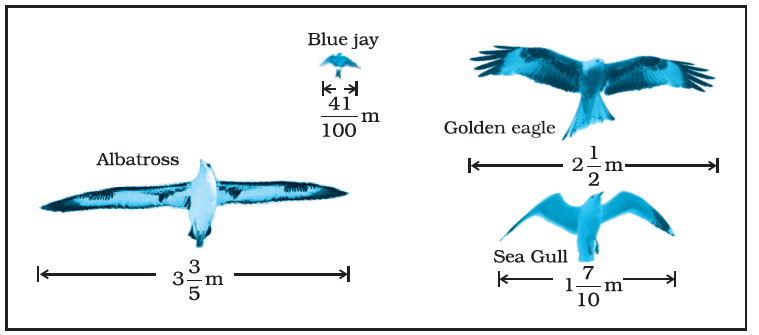(a) How much longer is the wingspan of an Albatross than the wingspan of a Sea gull?

Solution:-

We have to find out the difference of wingspan of an Albatross and wingspan of a Sea gull.

Length of wingspan of an Albatross =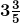= 18/5 m

Length of wingspan of a Sea gull =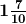= 17/10 m

Difference of both = (18/5) – (17/10)

= (36 – 17)/ 10

= 19/10 m

∴The wingspan of an Albatross is 19/10 m longer than the wingspan of a Sea gull.

(b) How much longer is the wingspan of a Golden eagle than the wingspan of a Blue jay?

Solution:-

We have to find out the difference of wingspan of a Golden eagle and wingspan of a Blue jay.

Length of wingspan of a Golden eagle = 2½ = 5/2 m

Length of wingspan of a Blue jay = 41/100 m

Difference of both = (5/2) – (41/100)

= (250 – 41)/ 100

= 209/100 m

∴The wingspan of a Golden eagle is 209/100 m longer than the wingspan of a Blue jay.

142. Shalini has to cut out circles of diameter 1¼ cm from an aluminum strip of dimensions 8¾ cm by 1¼ cm. How many full circles can Shalini cut? Also calculate the wastage of the aluminum strip.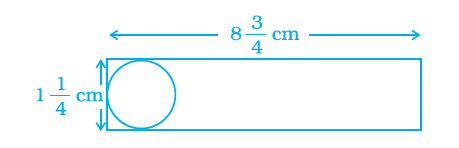Solution:-

From the question it is given that,

Diameter of the circle = Breadth of the aluminium strip 1¼ cm = 5/4 cm

Length of aluminium strip = 8¾ cm = 35/4 cm

∴The number of full circles cut from the aluminum strip = (35/4) ÷ (5/4)

= (35/4) × (4/5)

= (7/1) × (1/1)

= 7 circles

Radius of circle = (5/ (4 × 2)) = 5/8 cm

Area to be cut by one circle = πr2

= (22/7) × (5/8)2

= (22/7) × (25/64) cm2

Now, area to be cut by 7 full circles = 7 × (22/7) × (25/64)

= (22 × 25)/64

= 550/64 cm2

Area of the aluminum strip = length × breadth

= (35/4) × (5/4) cm2

= (175/16) cm2

∴The wastage of aluminum strip = (175/16) – (550/64)

= (700 – 550)/64

= 150/64

= 75/32 cm2

143. One fruit salad recipe requires ½ cup of sugar. Another recipe for the same fruit salad requires 2 tablespoons of sugar. If 1 tablespoon is equivalent to 1/16 cup, how much more sugar does the first recipe require?

Solution:-

From the question it is given that,

One fruit salad recipe requires = ½ cup of sugar

Sugar required for another salad = 2 × (1/16) = 2/16 cup

Hence, the required sugar = ½ – (2/16)

= (8 – 2)/16

= 6/16

= 3/8 cup of sugar.

144. Four friends had a competition to see how far could they hop on one foot. The table given shows the distance covered by each.

 Name Distance covered (km) Seema 1/25 Nancy 1/32 Megha 1/40 Soni 1/20

(a) How farther did Soni hop than Nancy?

(b) What is the total distance covered by Seema and Megha?

(c) Who walked farther, Nancy or Megha?

Solution:-

The LCM of the denominators 25, 32, 40 and 20 is 800

∴ 1/25 = [(1×32)/ (25×32)] = (32/800)

(1/32) = [(1×25)/ (32×25)] = (25/800)

(1/40) = [(1×20)/ (40×20)] = (20/800)

(1/20) = [(1×40)/ (20×40)] = (40/800)

Then,

(a) Soni hop more than Nancy = (40/800) – (25/800)

= (40 – 25)/800

= (15/800)

= 3/160 km

(b) The total distance covered by Seema and Megha = (32/800) + (20/800)

= (32 + 20)/800

= (52/800)

= 13/200 km

(c) Nancy walked farther.

145. The table given below shows the distances, in kilometers, between four villages of a state. To find the distance between two villages, locate the square where the row for one village and the column for the other village intersect.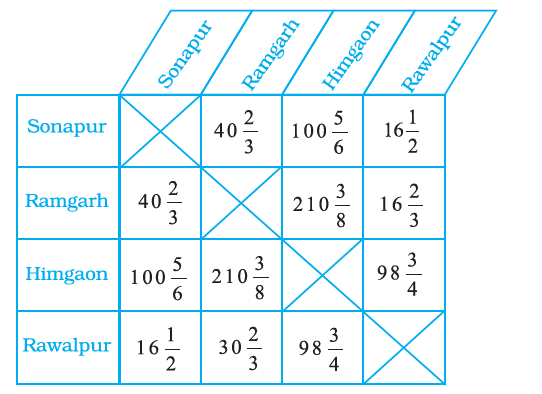(a) Compare the distance between Himgaon and Rawalpur to Sonapur and Ramgarh?

Solution:-

From the table the distance between Himgaon and Rawalpur = 98¾ km = 395/4 km

The distance between Sonapur and Ramgarh =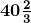= 122/3 km

Then,

Difference of the distance between Himgaon and Rawalpur to Sonapur and Ramgarh,

= ((395/4) – (122/3))

= (1185 – 488)/ 12

= 697/12

=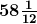km

(b) If you drove from Himgaon to Sonapur and then from Sonapur to Rawalpur, how far would you drive?

Solution:-

From the table,

Distance between Himgaon and Sonapur =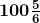km = 605/6 km

Distance between Sonapur and Rawalpur = 16 ½ km = 33/2

Then,

Total distance that he would drive,

= 605/6 + 33/2

= (605 + 99)/6

= 704/6

= 352/3

=km

146. The table shows the portion of some common materials that are recycled.

 Material Recycled Paper 5/11 Aluminium cans 5/8 Glass 2/5 Scrap ¾

(a) Is the rational number expressing the amount of paper recycled more than ½ or less than ½?

Solution:-

The rational number expressing the amount of paper recycled is less than ½.

(b) Which items have a recycled amount less than ½?

Solution:-

Paper and Glass have a recycled amount less than ½.

(c) Is the quantity of aluminium cans recycled more (or less) than half of the quantity of aluminium cans?

Solution:-

The quantity of aluminium cans recycled is more than half of the quantity of aluminium cans.

(d) Arrange the rate of recycling the materials from the greatest to the smallest.

Solution:-

The LCM of the denominators 11, 8, 5 and 4 is 440

∴ 5/11 = [(5×40)/ (11×40)] = (200/440)

(5/8) = [(5×55)/ (8×55)] = (275/440)

(2/5) = [(2×88)/ (5×88)] = (176/440)

(3/4) = [(3×110)/ (4×110)] = (330/440)

Then,

Now, 330 > 275 > 200 > 176

Hence, ⇒ 3/4 > 5/8 > 5/11 > 2/5

∴Scrap > Aluminium > Cans > Paper > Glass.

147. The overall width in cm of several wide-screen televisions are 97.28 cm,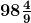cm,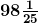cm and 97.94 cm. Express these numbers as rational numbers in the form p/q and arrange the widths in ascending order.

Solution:-

From the question,

The overall width in cm of several wide screen television are,

97.28 cm = 9728/100 … [∵by the decimal removing method]

By dividing both numerator and denominator by 4 we get,

= 2432/25cmcm = by converting mixed fraction into improper fraction we get,

= 886/9 cmcm = by converting mixed fraction into improper fraction we get,

= 2451/25 cm

97.94 cm = 9794/100 … [∵by the decimal removing method]

By dividing both numerator and denominator by 2 we get,

= 4897/50cm

Now, we have to take the LCM of denominators to arrange them in ascending order.

The LCM of the denominators 25, 9, 25 and 50 is 450

∴ 2432/25= [(2432×18)/ (25×18)] = (43776/450)

(886/9) = [(886×50)/ (9×50)] = (44300/450)

(2451/25) = [(2451×18)/ (25×18)] = (44118/450)

(4897/50) = [(4897×9)/ (50×9)] = (44073/450)

Then,

Now, 43776 < 44073 < 44118 < 44300

Hence, in ascending order = (2432/25) < (4897/50) < (2451/25) < (886/9)

∴97.28 < 97.94 <cm <cm

148. Roller Coaster at an amusement park is 2/3m high. If a new roller coaster is built that is 3/5 times the height of the existing coaster, what will be the height of the new roller coaster?

Solution:-

From the question it is given that,

Height of the roller coaster at an amusement park = 2/3 m

Height of the new roller coaster is about to build = 3/5 times the height of the existing

Coaster

= (2/3) × (3/5)

= (2/1) × (1/5)

= (2/5) m

149. Here is a table which gives the information about the total rainfall for several months compared to the average monthly rains of a town. Write each decimal in the form of rational number p/q.

 Month Above/Below normal (in cm) May 2.6924 June 0.6096 July -6.9088 August -8.636

Solution:-

(i) May

2.6924 cm = 26924/10000 … [∵by the decimal removing method]

By dividing both numerator and denominator by 4 we get,

= 6731/2500 cm

(ii) June

0.6096 cm = 0.6096/10000 … [∵by the decimal removing method]

By dividing both numerator and denominator by 16 we get,

= 381/625 cm

(iii) July

-6.9088 cm = -69088/10000 … [∵by the decimal removing method]

By dividing both numerator and denominator by 4 we get,

= -4318/625 cm

(iv)August

-8.636 cm = -8636/1000 … [∵by the decimal removing method]

By dividing both numerator and denominator by 4 we get,

= -2159/250 cm

150. The average life expectancies of males for several states are shown in the table. Express each decimal in the form p/q and arrange the states from the least to the greatest male life expectancy. State-wise data are included below; more indicators can be found in the “FACTFILE” section on the homepage for each state.

 State Male p/q form Lowest terms Andhra Pradesh 61.6 Assam 57.1 Bihar 60.7 Gujarat 61.9 Haryana 64.1 Himachal Pradesh 65.1 Karnataka 62.4 Kerala 70.6 Madhya Pradesh 56.5 Maharashtra 64.5 Orissa 57.6 Punjab 66.9 Rajasthan 59.8 Tamil Nadu 63.7 Uttar Pradesh 58.9 West Bengal 62.8 India 60.8

Source: Registrar General of India (2003) SRS Based Abridged Lefe Tables. SRS Analytical Studies, Report No. 3 of 2003, New Delhi: Registrar General of India. The data are for the 1995-99 period; states subsequently divided are therefore included in their pre-partition states (Chhatisgarh in MP, Uttaranchal in UP and Jharkhand in Bihar)

Solution:-

 State Male p/q form Lowest terms Andhra Pradesh 61.6 616/10 308/5 Assam 57.1 571/10 571/10 Bihar 60.7 607/10 607/10 Gujarat 61.9 619/10 619/10 Haryana 64.1 641/10 641/10 Himachal Pradesh 65.1 651/10 651/10 Karnataka 62.4 624/10 312/5 Kerala 70.6 706/10 353/5 Madhya Pradesh 56.5 565/10 113/2 Maharashtra 64.5 645/10 129/2 Orissa 57.6 576/10 288/5 Punjab 66.9 669/10 669/10 Rajasthan 59.8 598/10 299/5 Tamil Nadu 63.7 637/10 637/10 Uttar Pradesh 58.9 589/10 589/10 West Bengal 62.8 628/10 314/5 India 60.8 608/10 304/5

Kerala; Punjab; HP; Maharashtra; Haryana; Tamil Nadu; West Bengal; Karnataka; Gujarat; Andhra Pradesh; Bihar; Rajasthan; UP; Orissa; Assam; MP

151. A skirt that is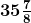cm long has a hem of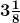cm. How long will the skirt be if the hem is let down?

Solution:-

From the question it is given that,

Length of the skirt =cm = 287/8 cm

Dimension of hem =cm = 25/8 cm

Length of skirt, if hem is let down = ((287/8) + (25/8)) cm

= 312/8 cm

= 39 cm

152. Manavi and Kuber each receives an equal allowance. The table shows the fraction of their allowance each deposits into his/her saving account and the fraction each spends at the mall. If allowance of each is ₹ 1260 find the amount left with each.

Solution:-

 Where money goes Fraction of allowance Manavi Kuber Saving Account ½ 1/3 Spend at mall ¼ 3/5 Left over ? ?

Solution:-

From the question,

Manavi and Kuber each receives and equal allowance = ₹ 1260

Let us assume total cost be ₹ 1

For Manavi, left over = Total cost – Total spends

= 1 – (½ + ¼)

= 1 – (2 + 1)/4

= 1 – (3/4)

= (4 – 3)/4

= ¼

So, Amount = 1260 × ¼ = ₹ 315

For Kuber, left over = Total cost – Total spends

= 1 – (1/3 + 3/5)

= 1 – (5 + 9)/15

= 1 – (14/15)

= (15 – 14)/15

= 1/15

So, Amount = 1260 × (1/15) = ₹ 84

Download BYJU’S – The Learning App and get personalised videos explaining Maths topics such as rational numbers, whole numbers, integers, etc., and experience a new way of learning to understand the concepts easily. To get exemplars for all the chapters of Maths Class 8, click here.

## Frequently Asked Questions on NCERT Exemplar Solutions for Class 8 Maths Chapter 1

Q1

### Why should we download NCERT Exemplar Solutions for Class 8 Maths Chapter 1?

The presentation of each solution in Chapter 1 of NCERT Exemplar Solutions for Class 8 Maths is described in a unique way by BYJU’S subject experts in Maths. The solutions are explained in understandable language, which improves conceptual understanding among students. To score good marks, practising NCERT Exemplar Solutions for Class 8 Maths can help to a great extent. This chapter can be used as a model of reference by students to improve their understanding of solving problems using different ways.
Q2

### Does BYJU’S website provide answers to NCERT Exemplar Solutions for Class 8 Maths Chapter 1 in a detailed way?

Yes, BYJU’S website provides answers to NCERT Exemplar Solutions for Class 8 Maths Chapter 1 in a step-by-step manner. This allows students to learn all concepts in detail, and they can also clear their doubts. Regular practising makes them score high in the Maths final exam.
Q3

### Give an overview of concepts present in NCERT Exemplar Solutions for Class 8 Maths Chapter 1.

The concepts of NCERT Exemplar Solutions for Class 8 Maths Chapter 1 are listed below.
1. Properties of rational numbers, whole numbers and integers
2. Closure, commutativity and associativity properties
3. Some more properties, such as the role of zero (0), the role of 1, the negative of a number and reciprocal
4. Distributivity of multiplication over addition for rational numbers
5. Number line representation of rational numbers
6. Finding rational numbers between two rational numbers